# West Bengal Madhyamik Board Question Paper for Class 10th Maths 2019 In PDF

West Bengal (Class 10) Maths 2019 question paper with solutions are provided on this page by BYJU’S in downloadable pdf format and also in the text, so that the students can equip themselves to face the West Bengal Madhyamik exam and score high marks. Students can solve and practise West Bengal (Class 10) Maths 2019 question paper, so that they can face the exam more confidently.

Maths Question Paper 2019 Class 10 is also added here, which helps students to get better acquainted with the important concepts that have to be covered during their preparation. Students are able to access all the West Bengal board previous year maths question papers here. Students can assess their abilities and problem-solving skills by practising different types of questions from the previous year papers and also predict the questions that are important and carry more weightage in the board exams.

### WBBSE Class 10th Maths Question Paper With Solutions 2019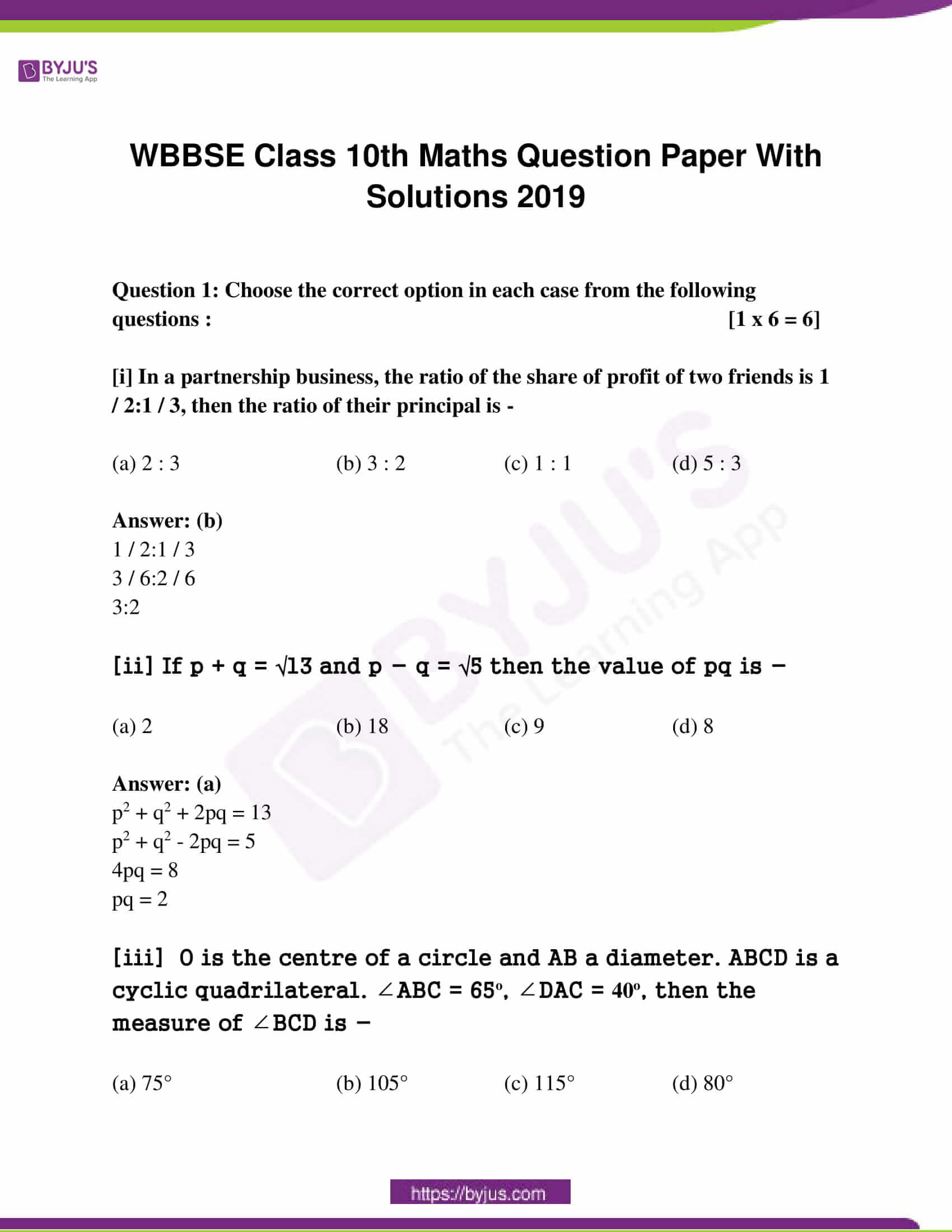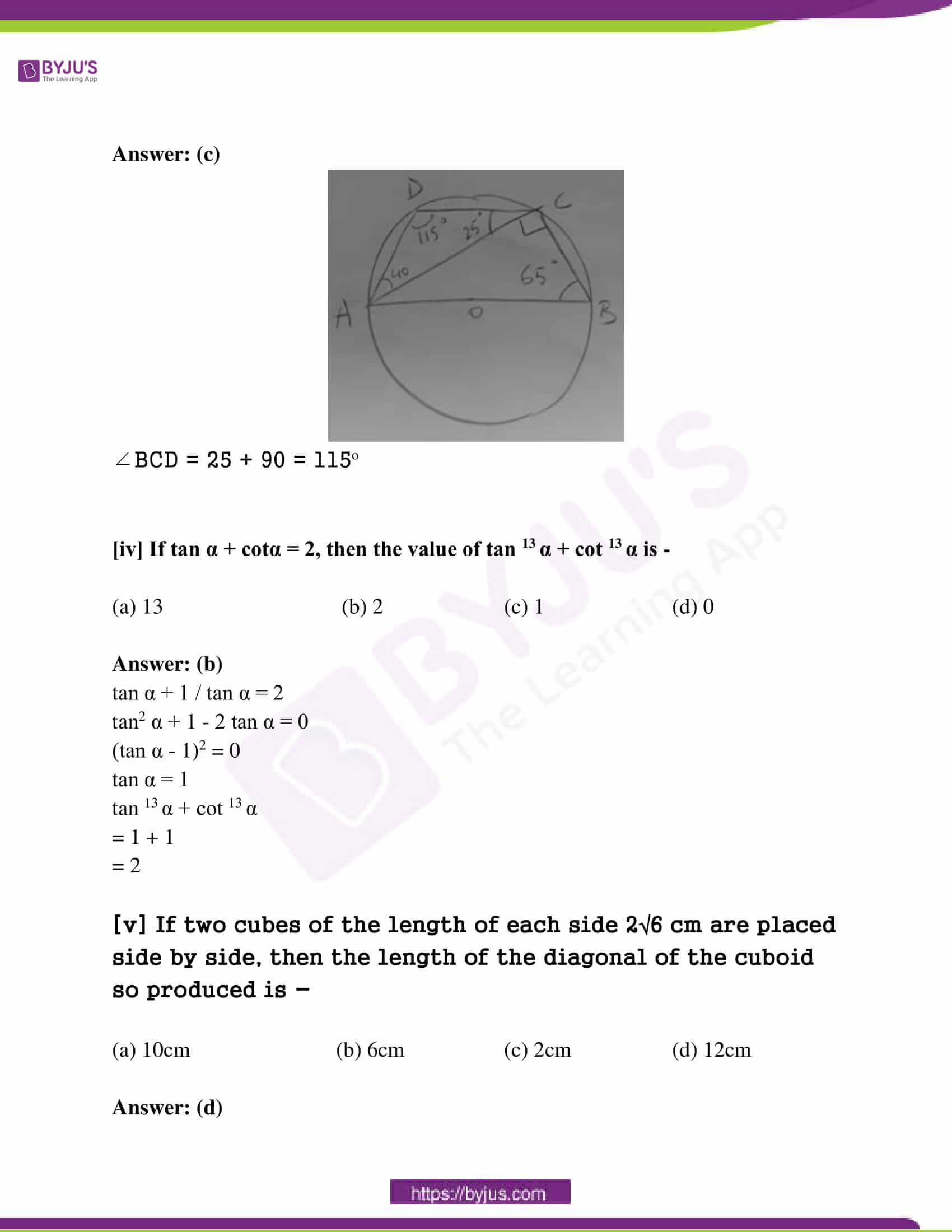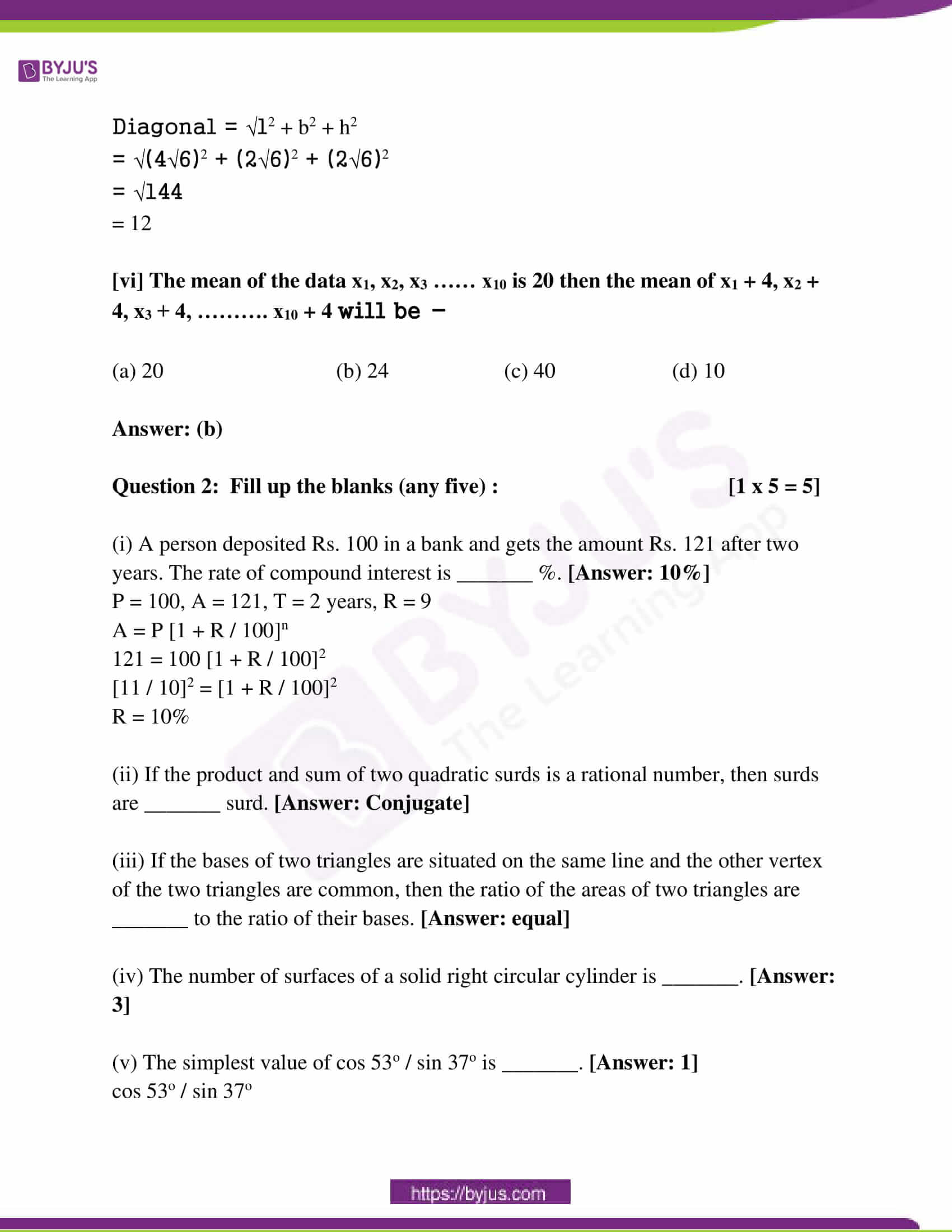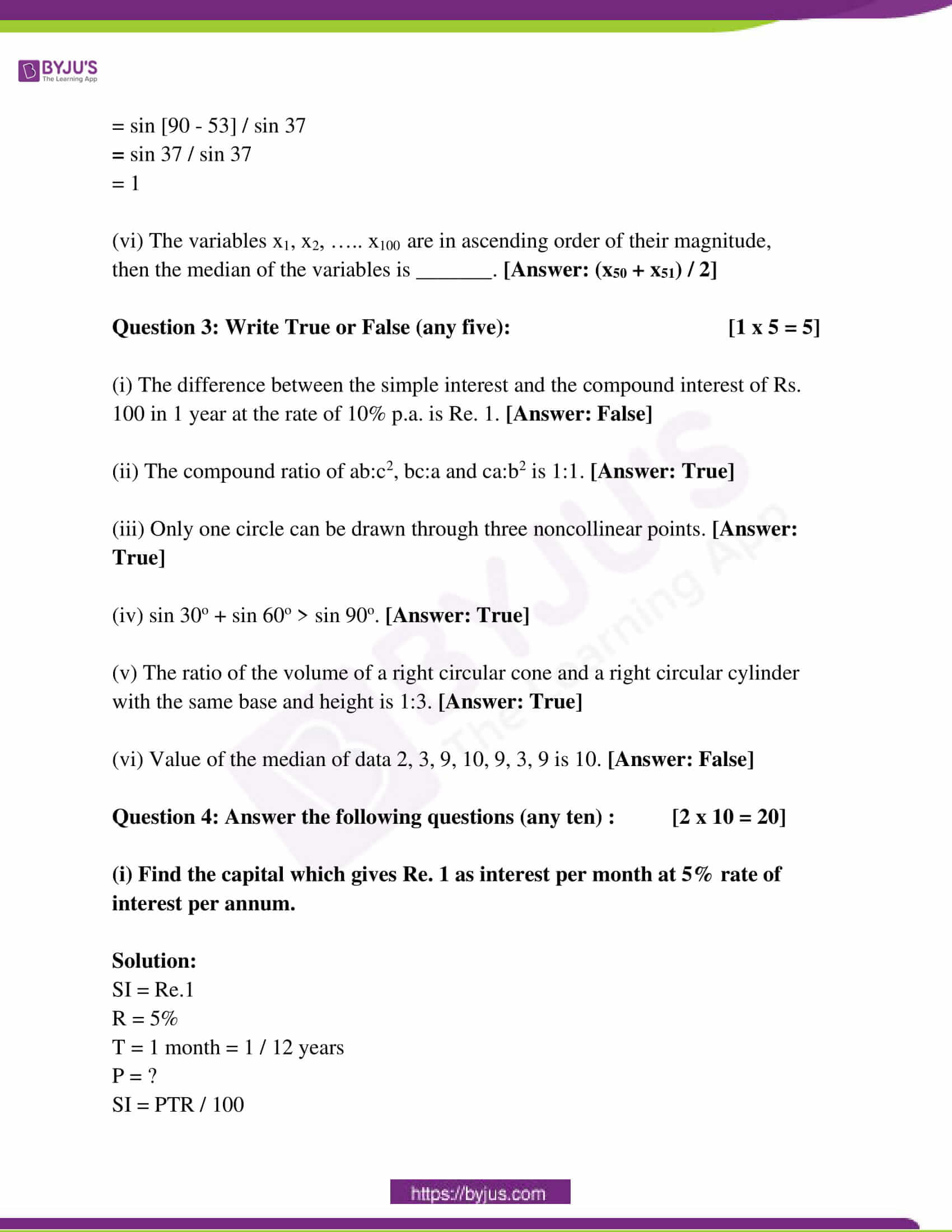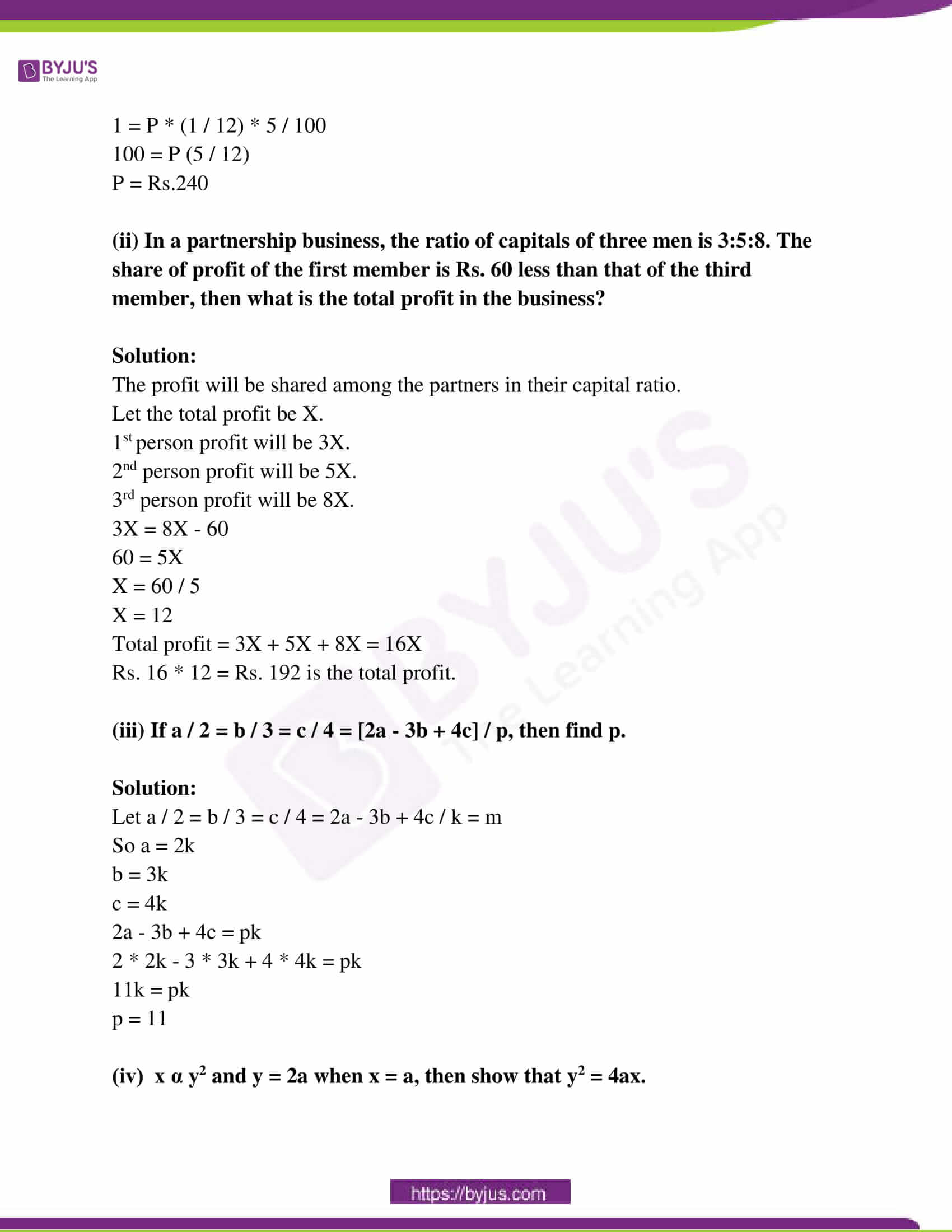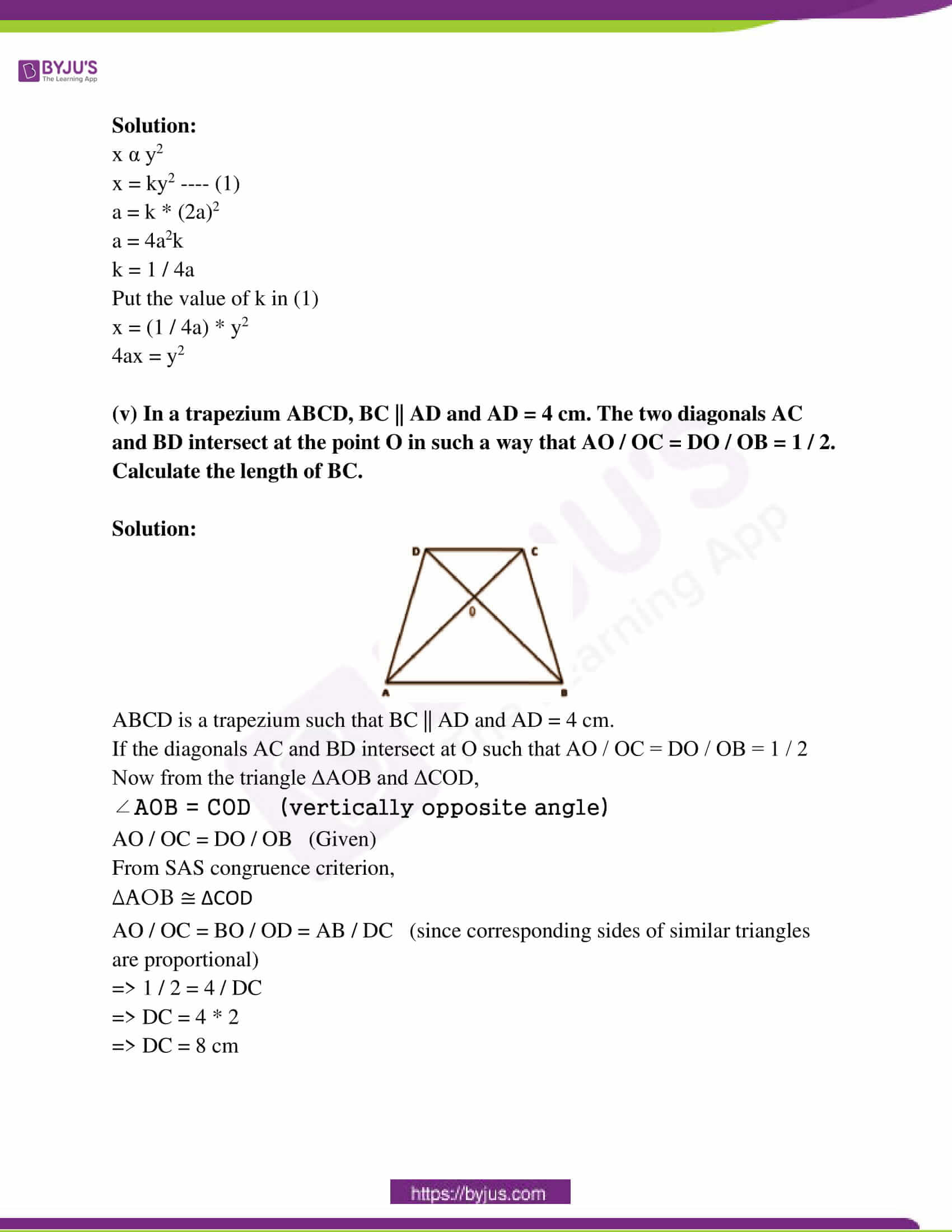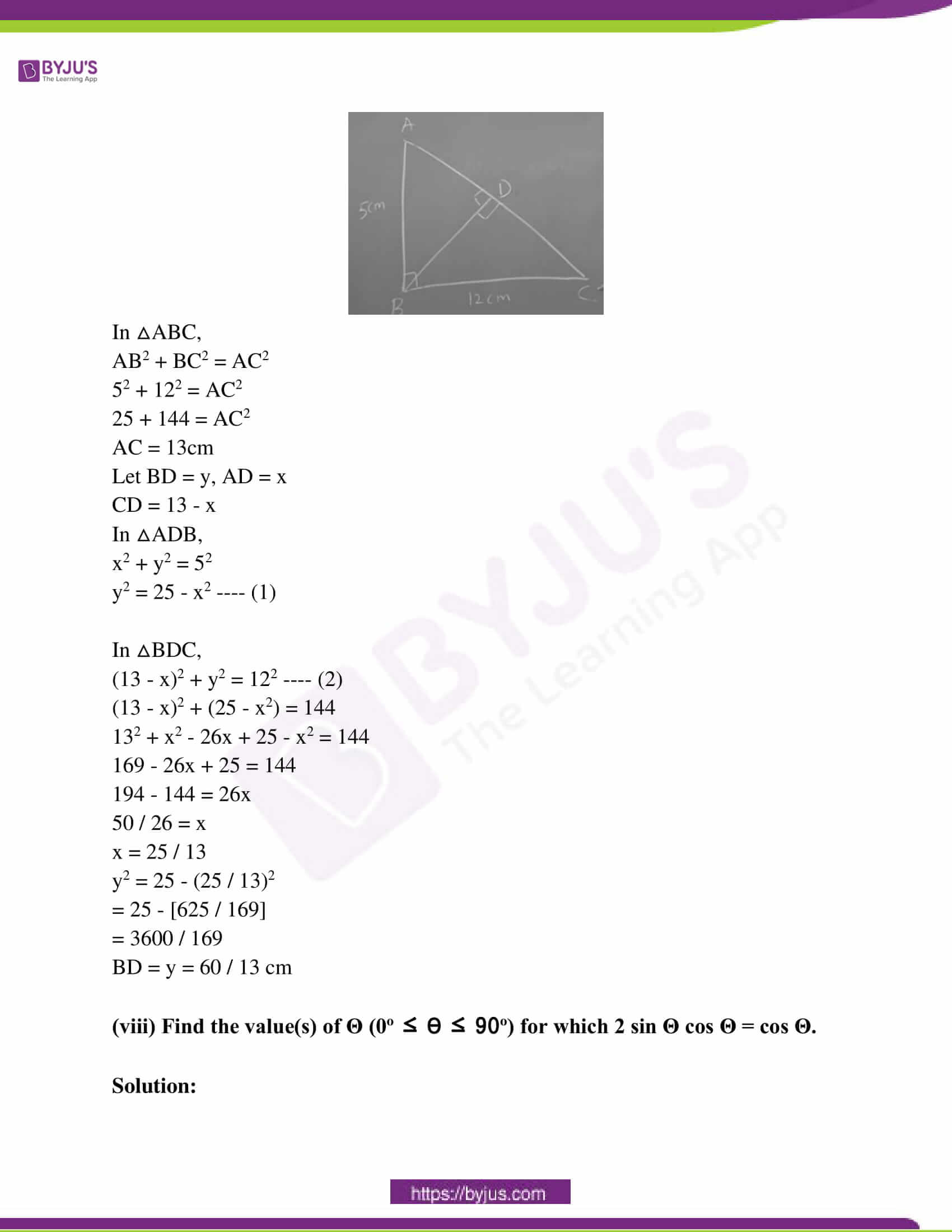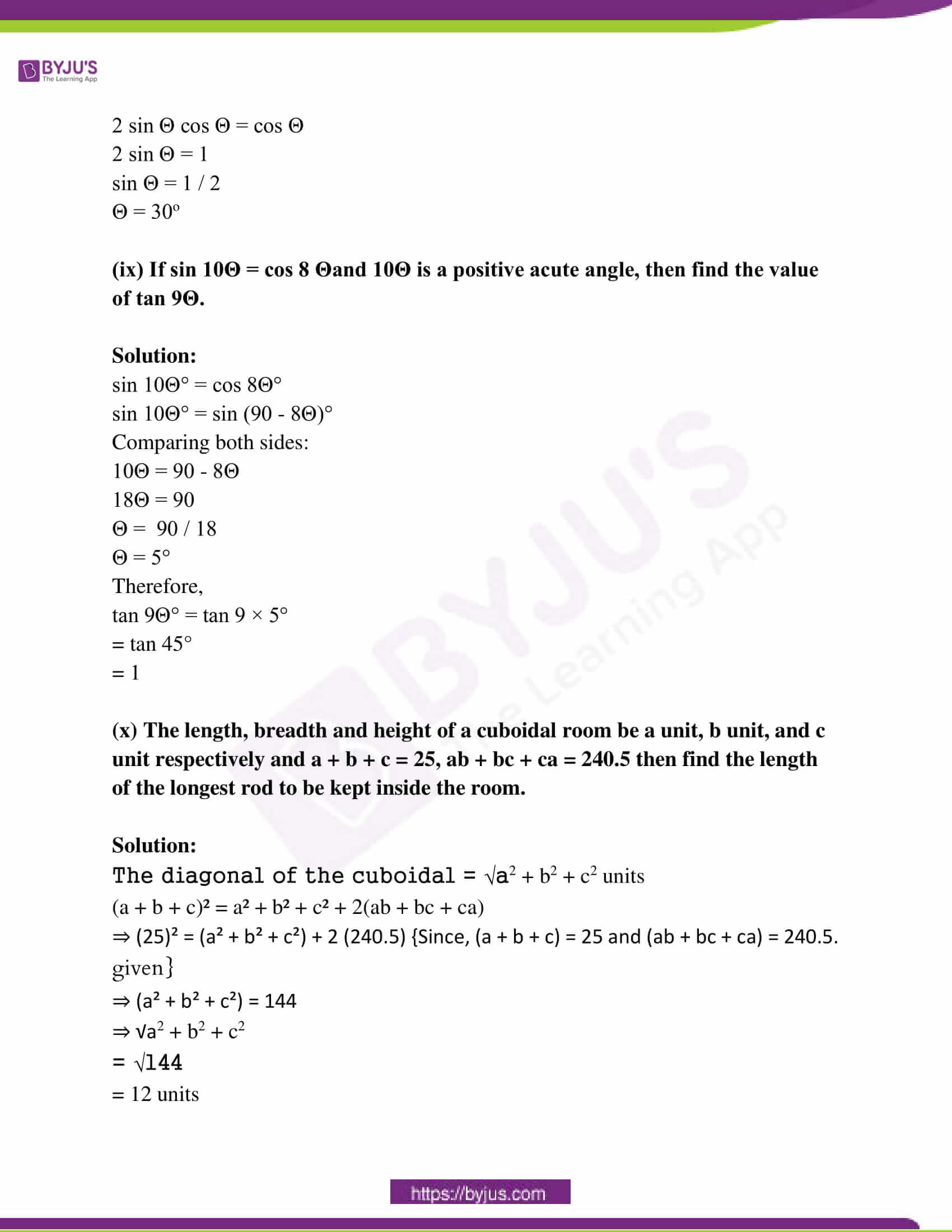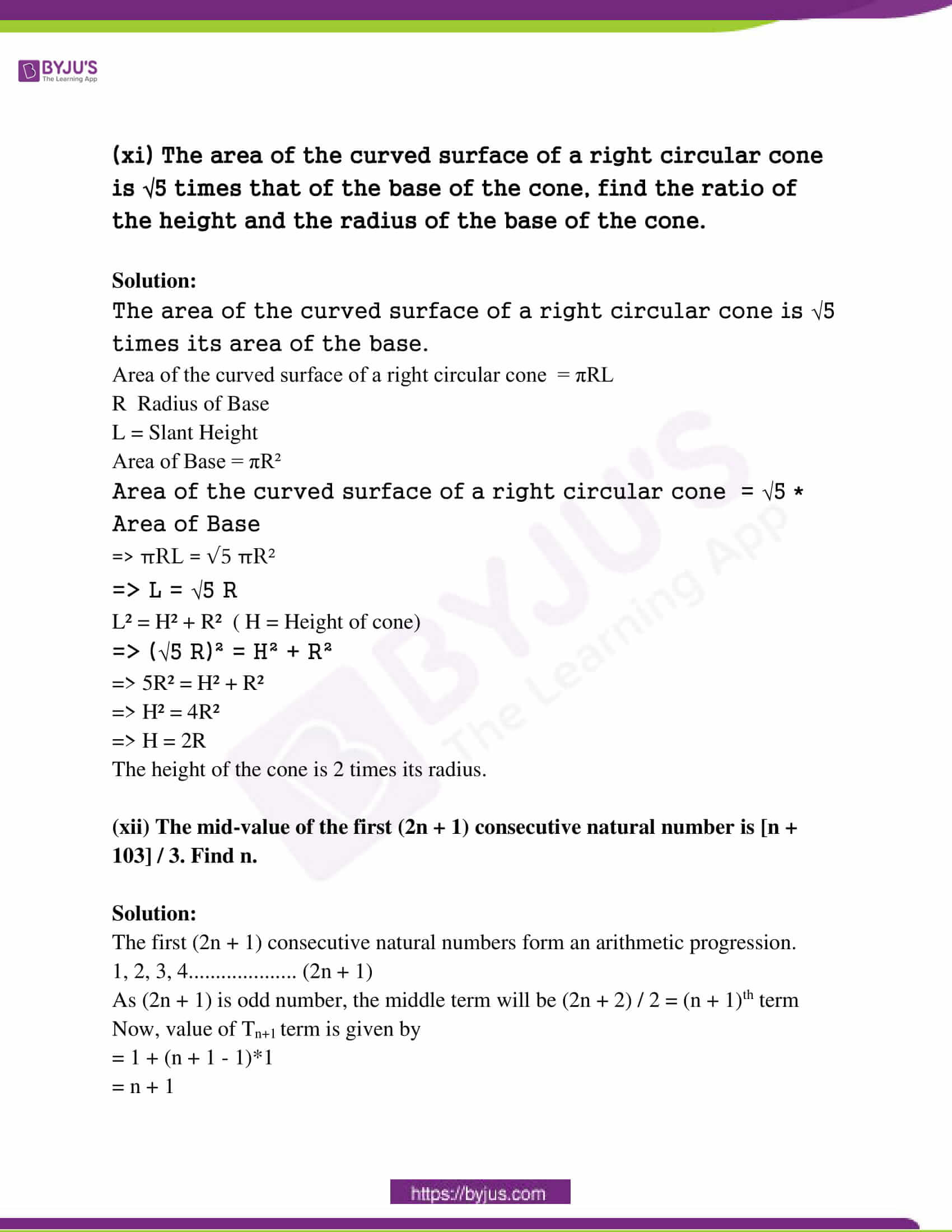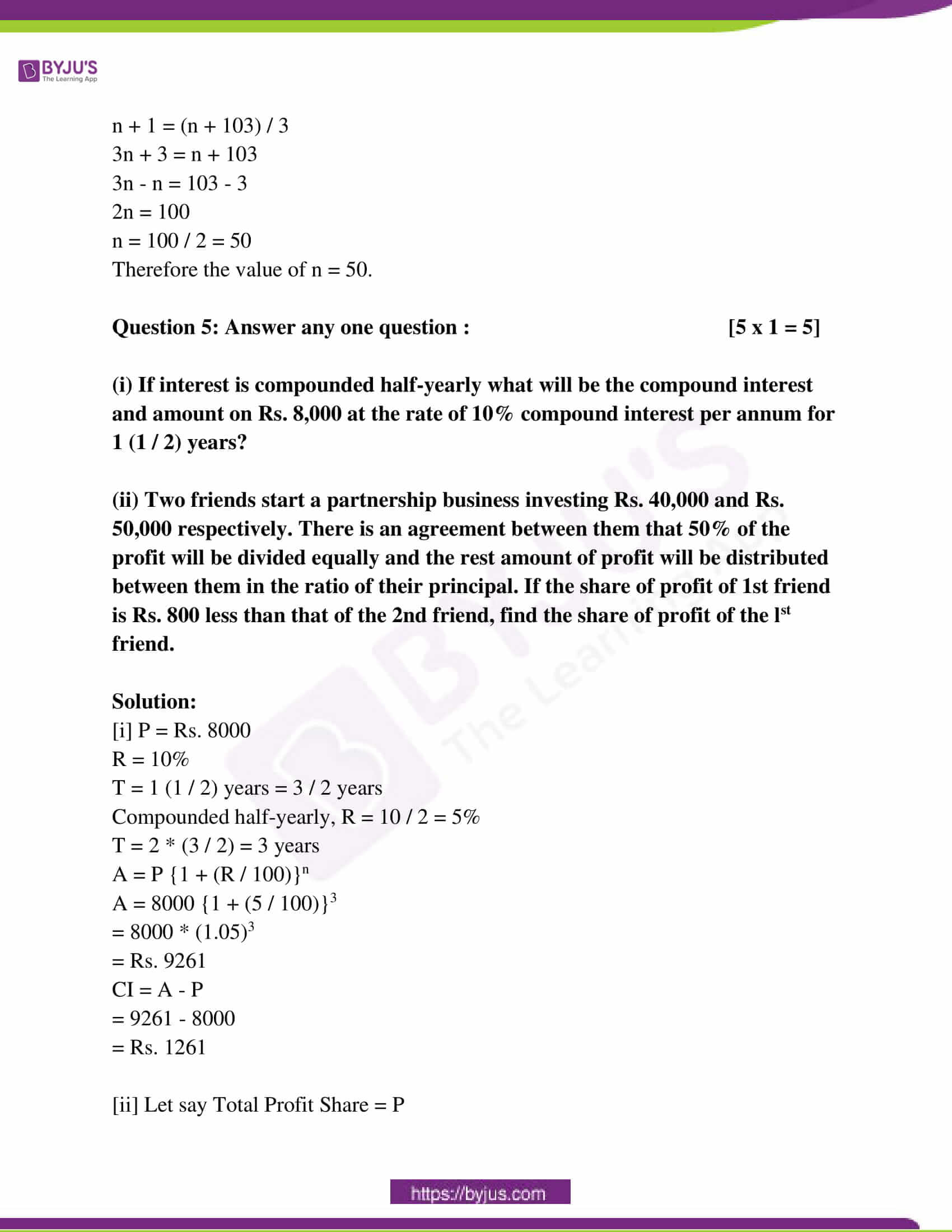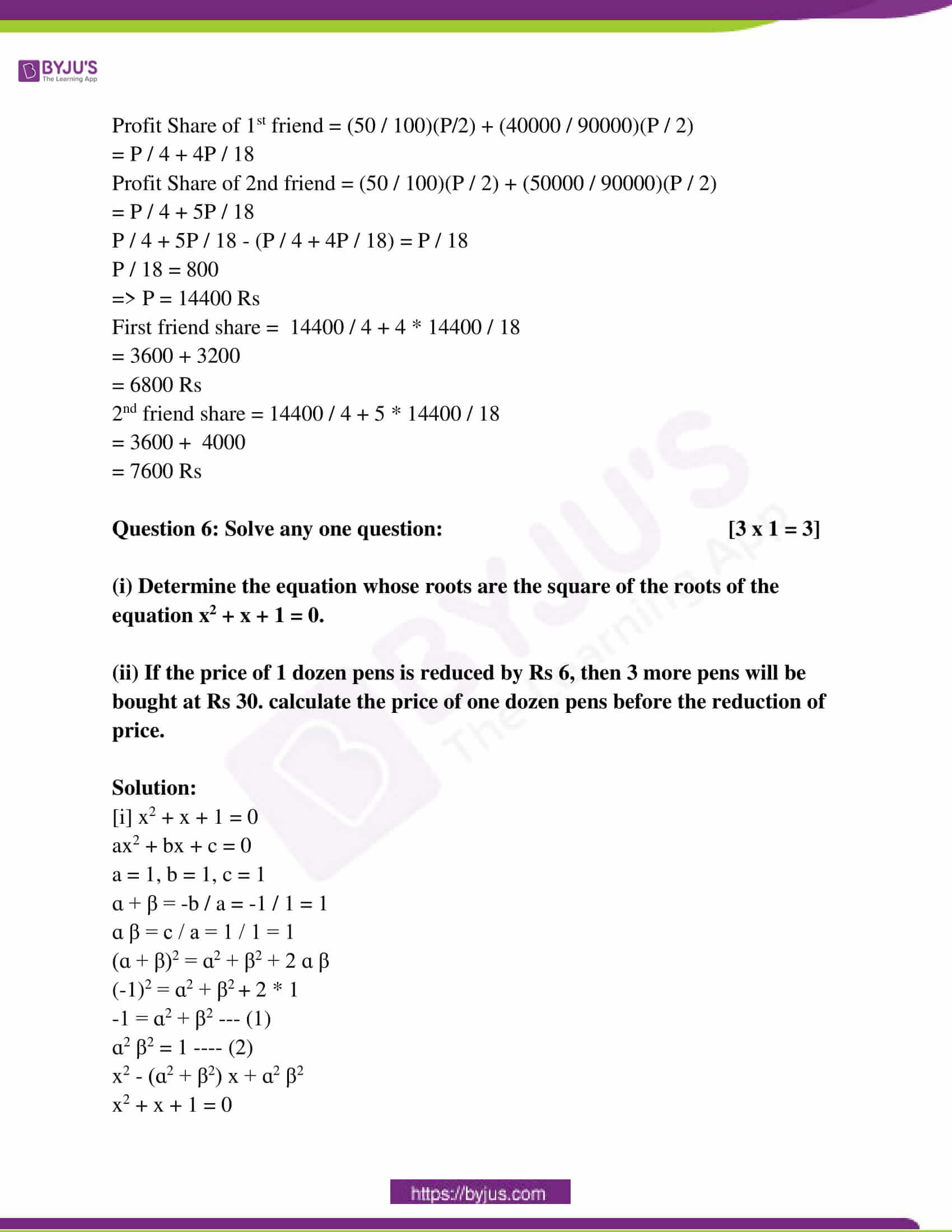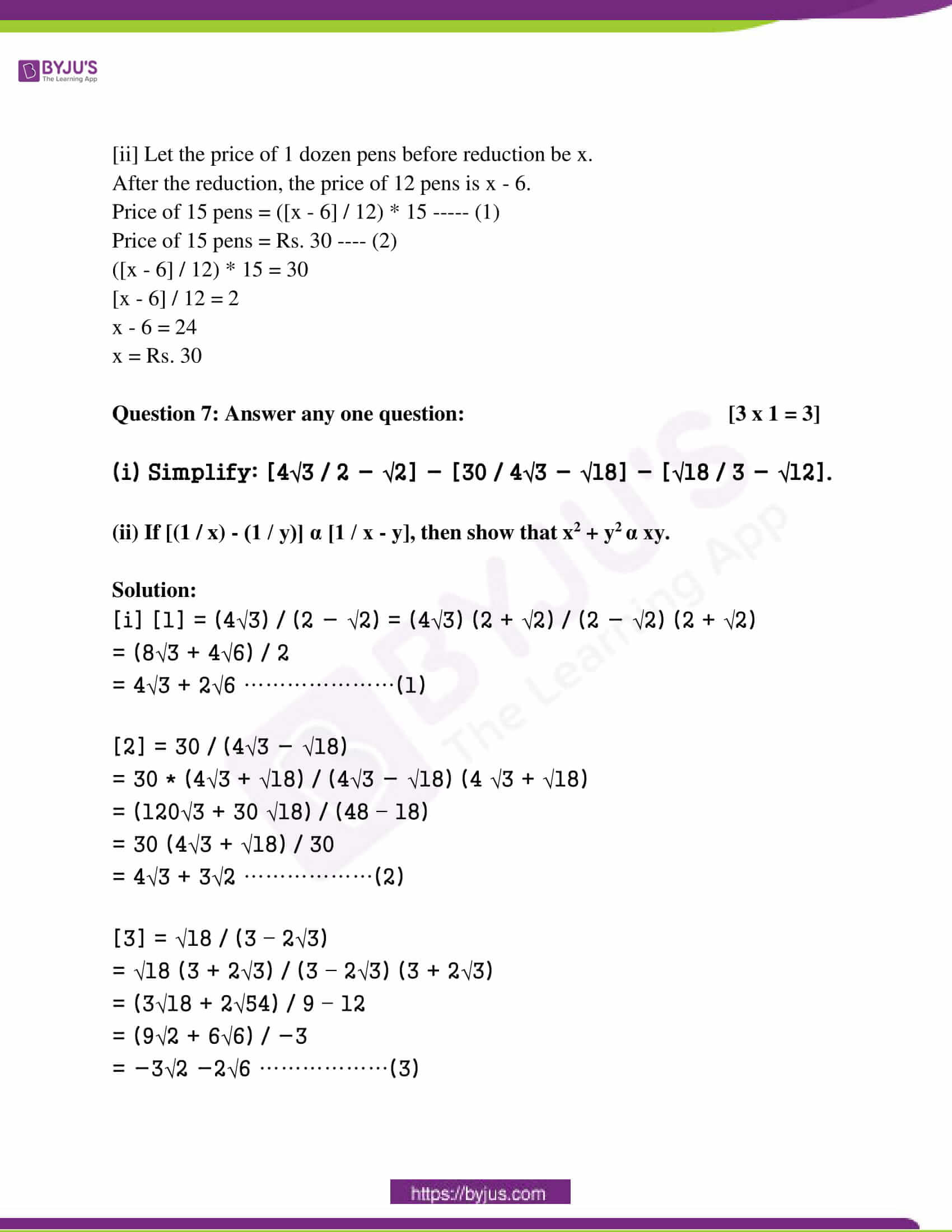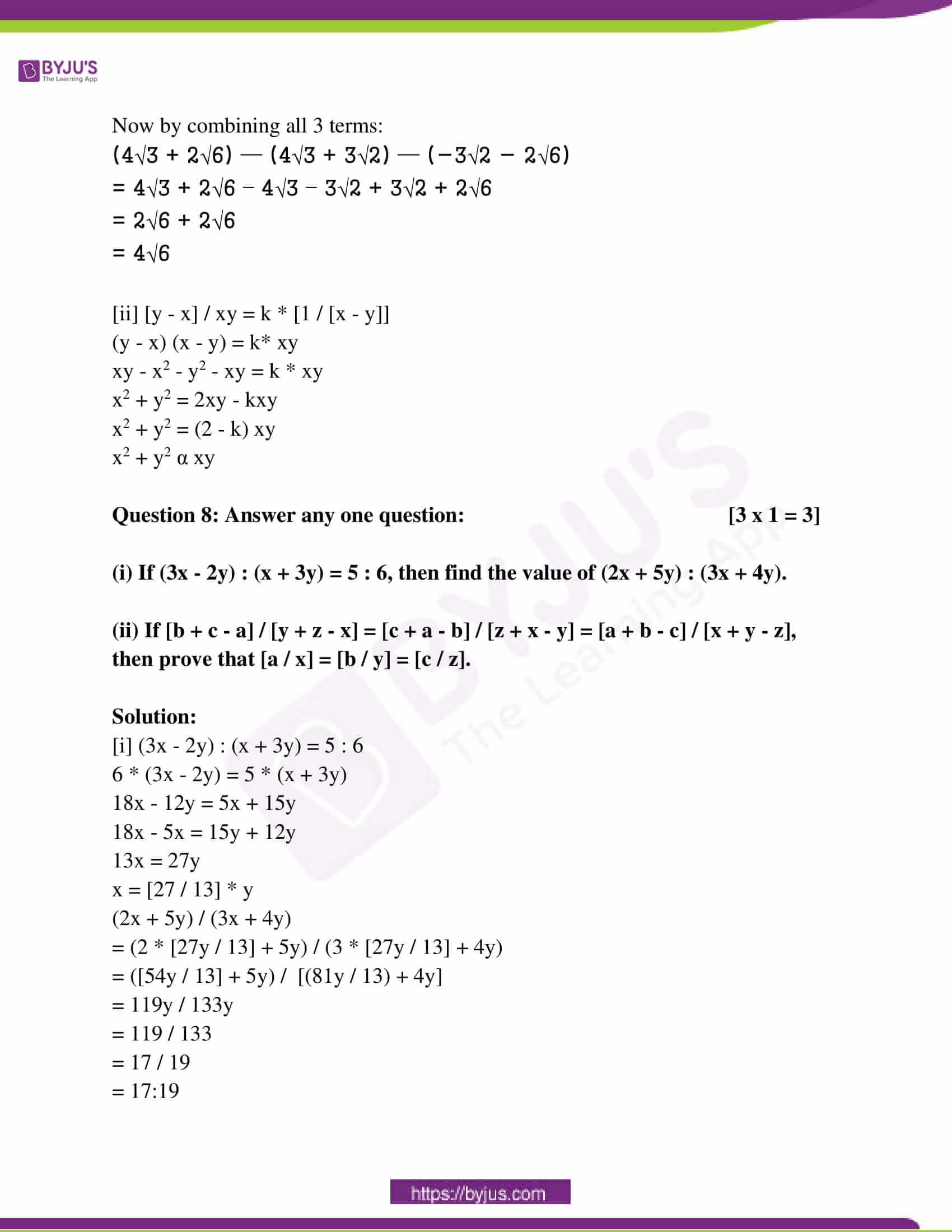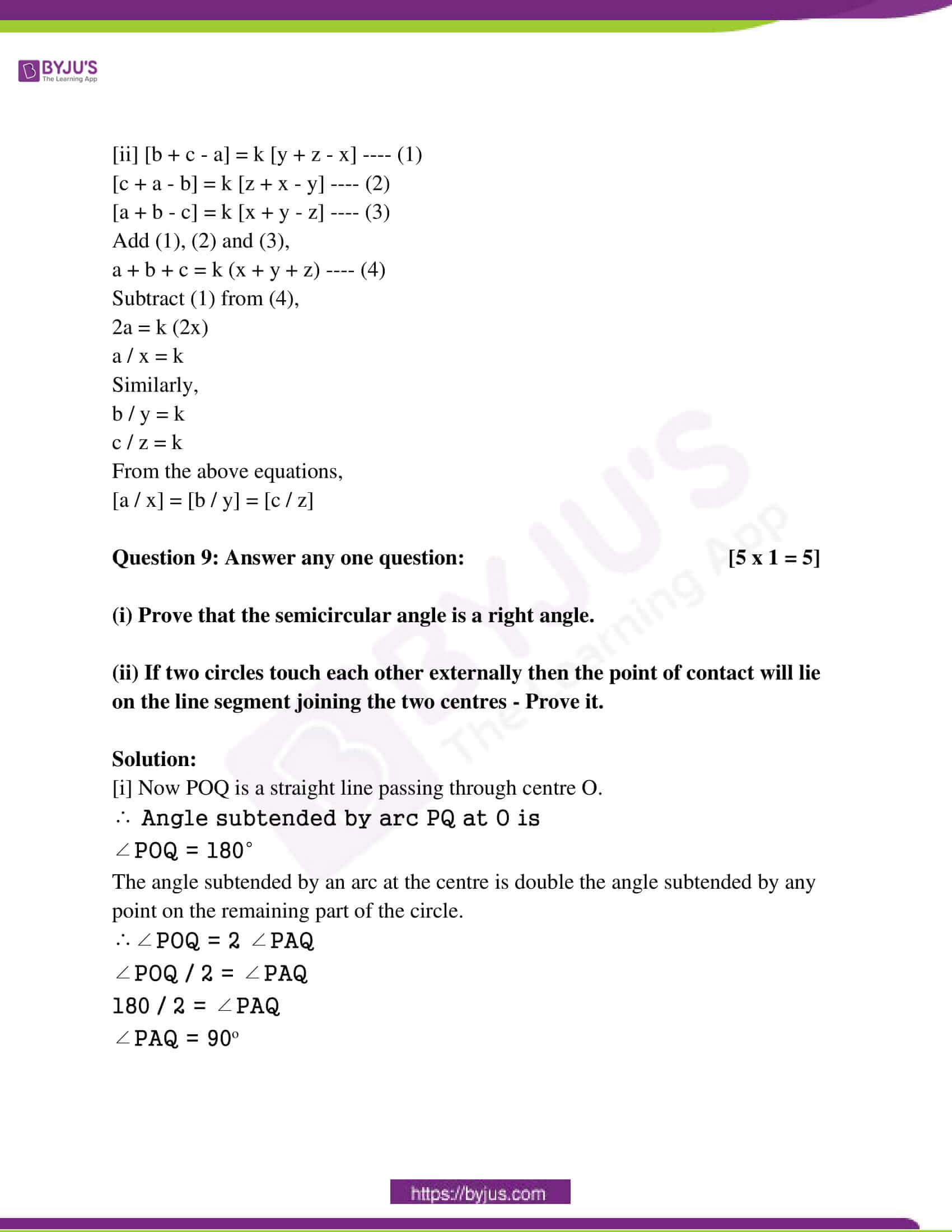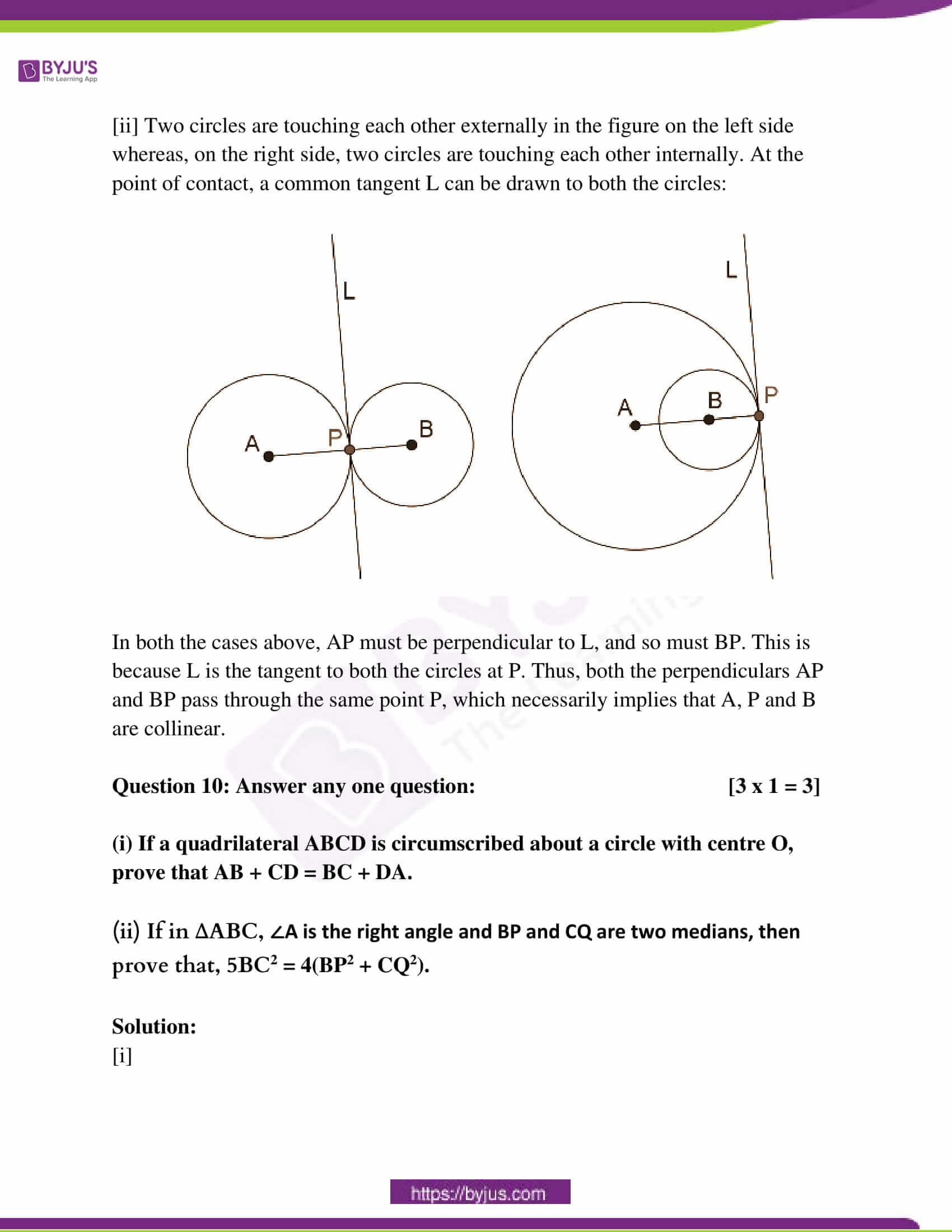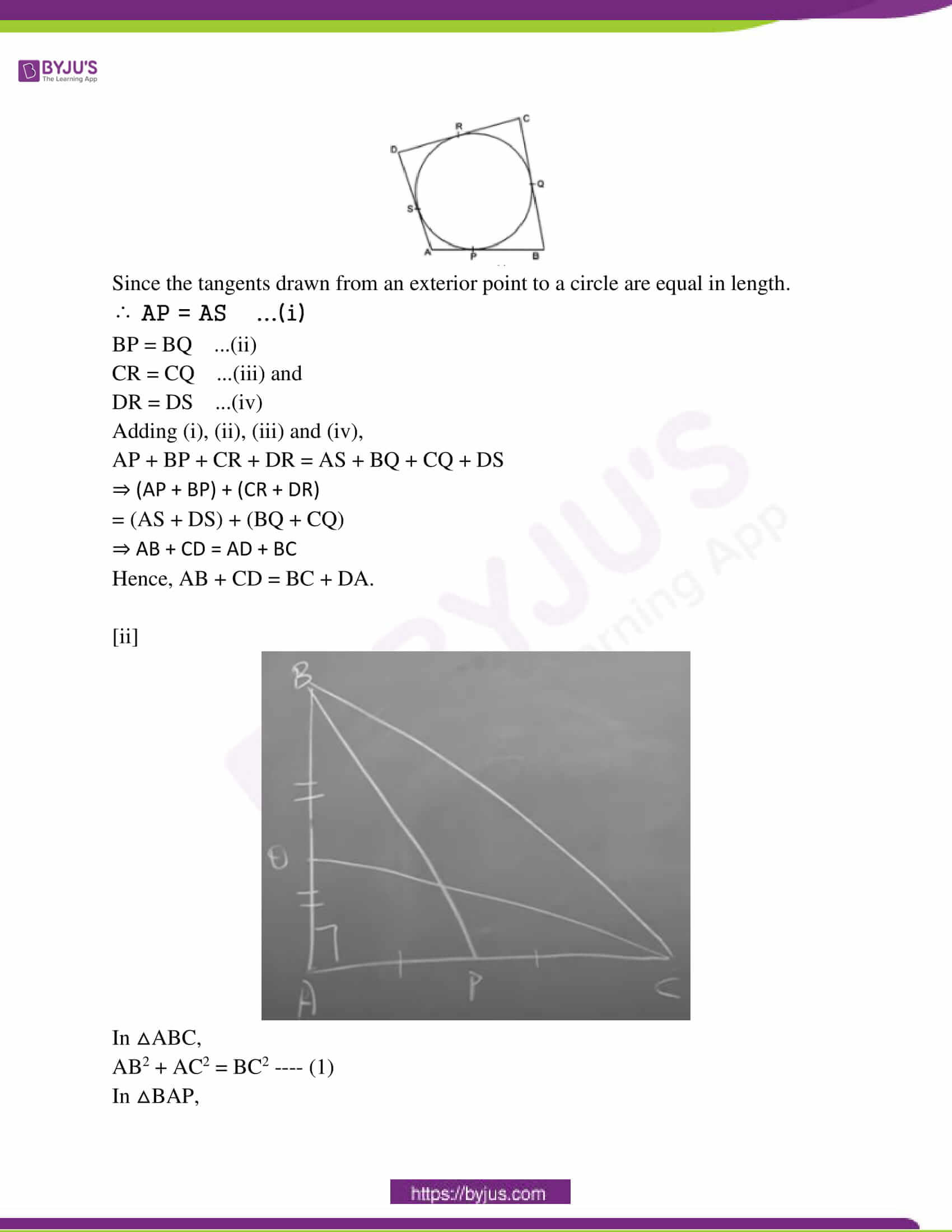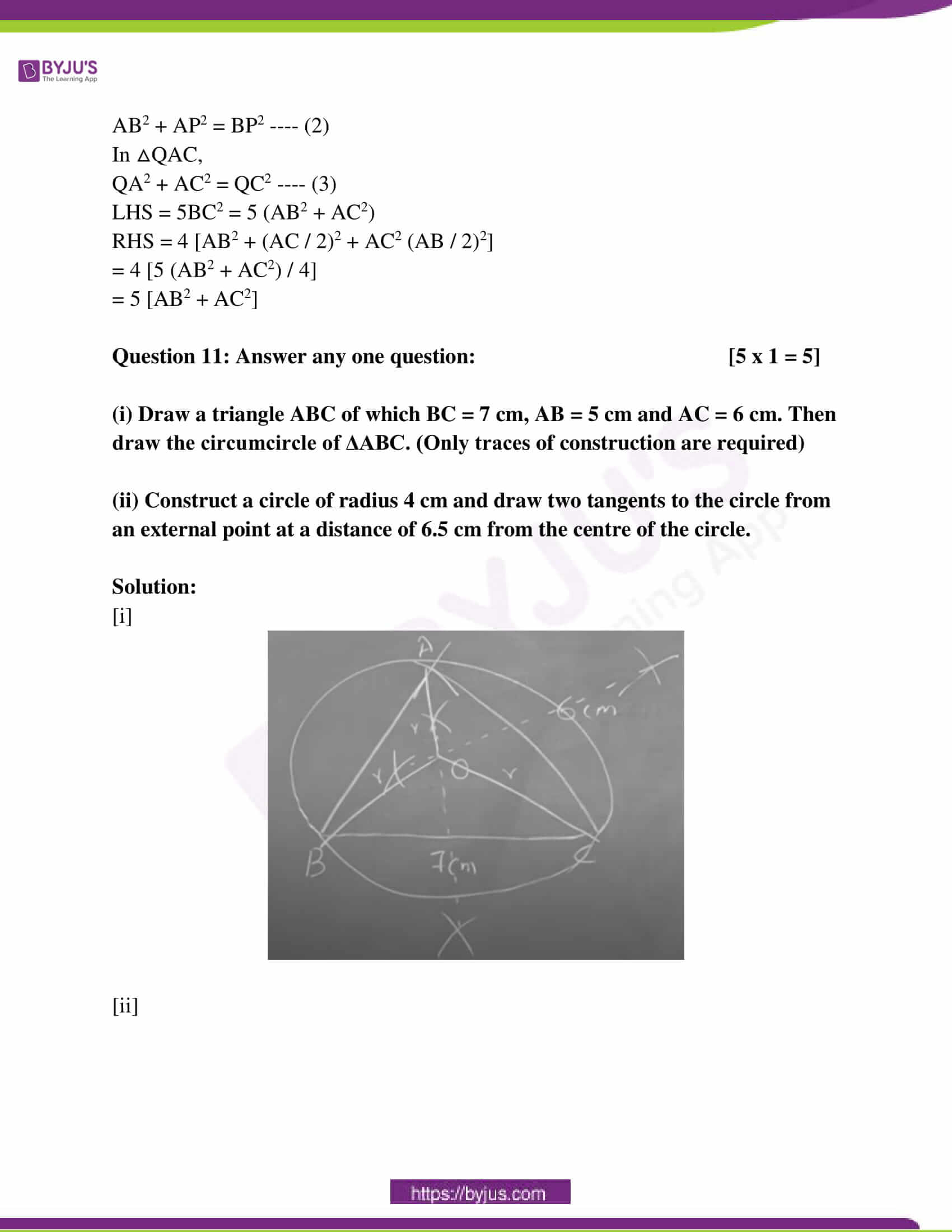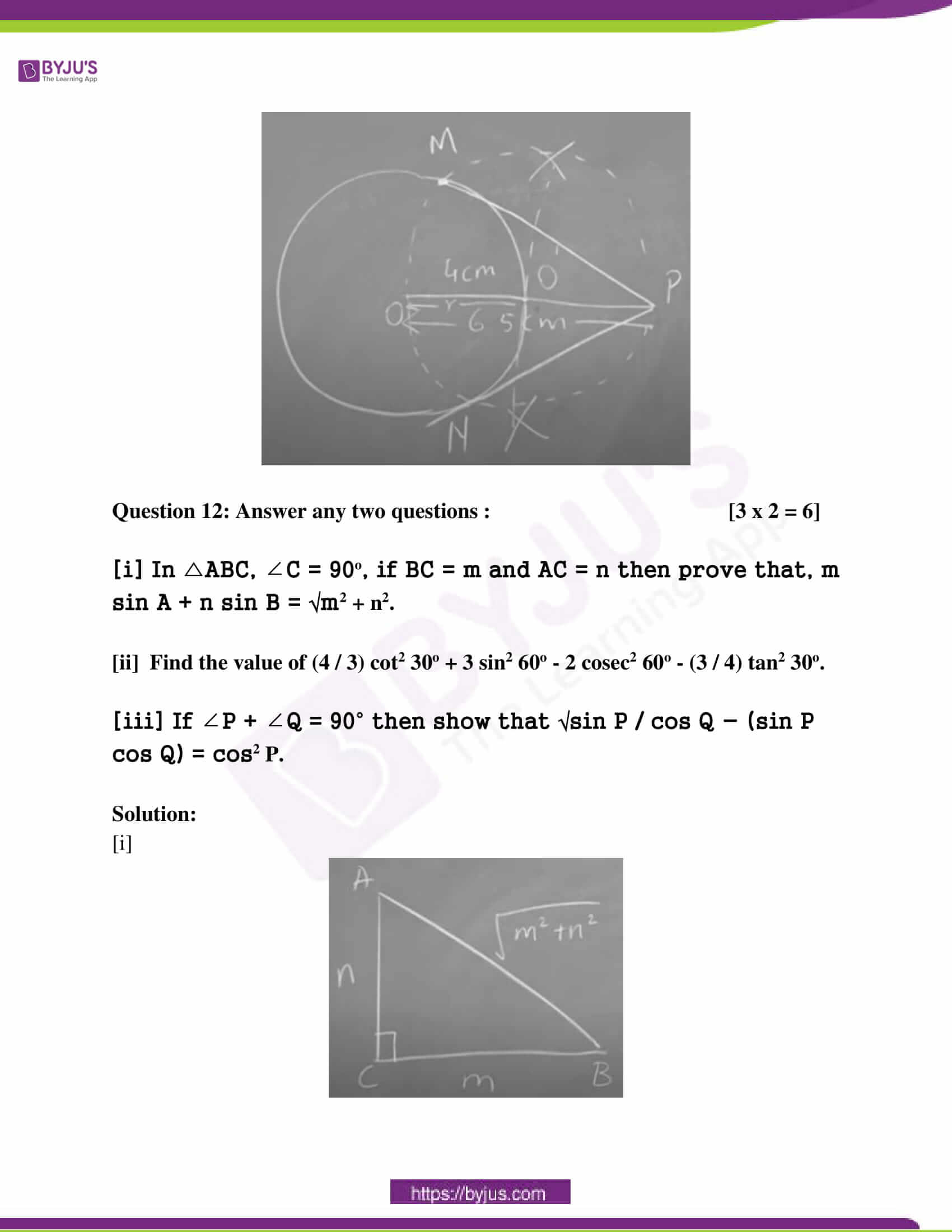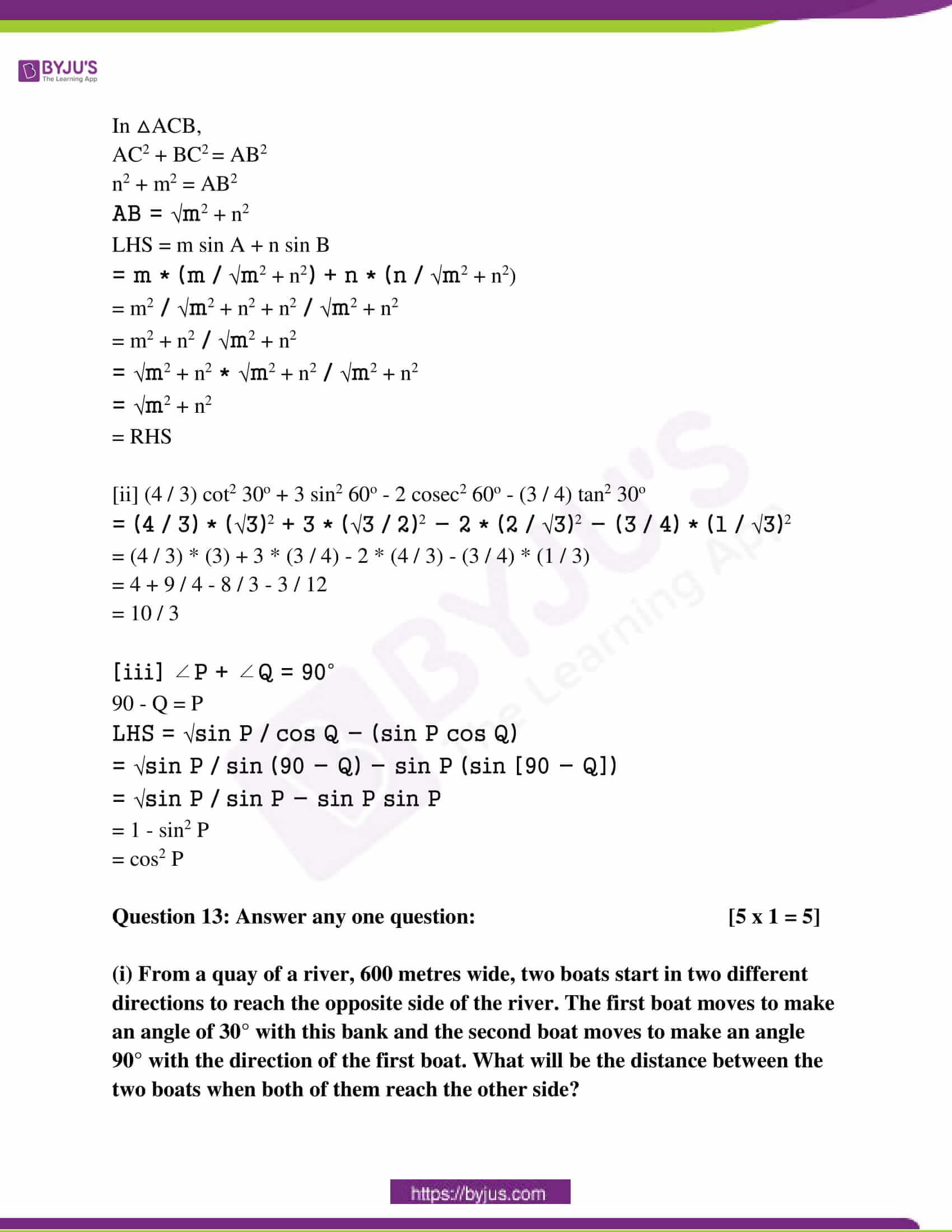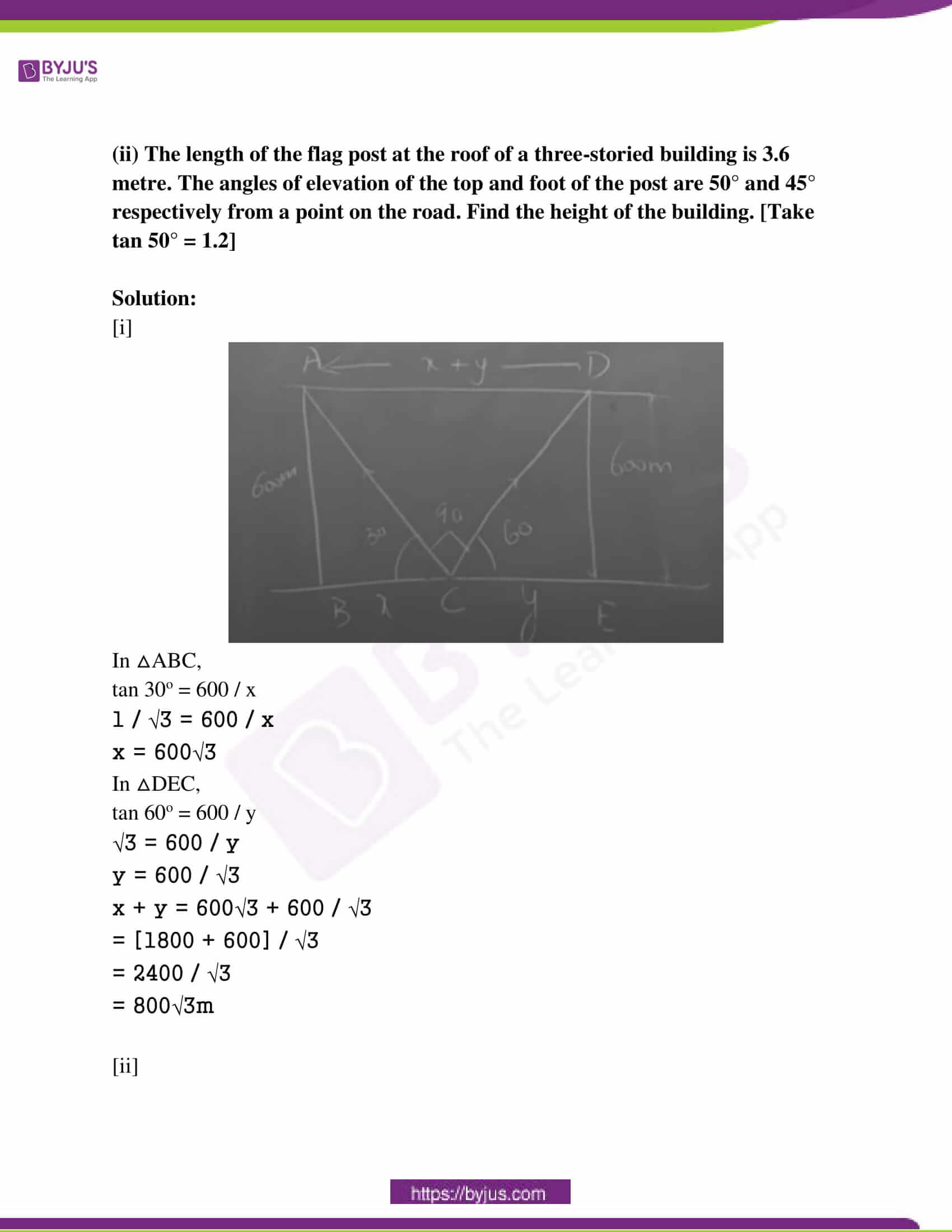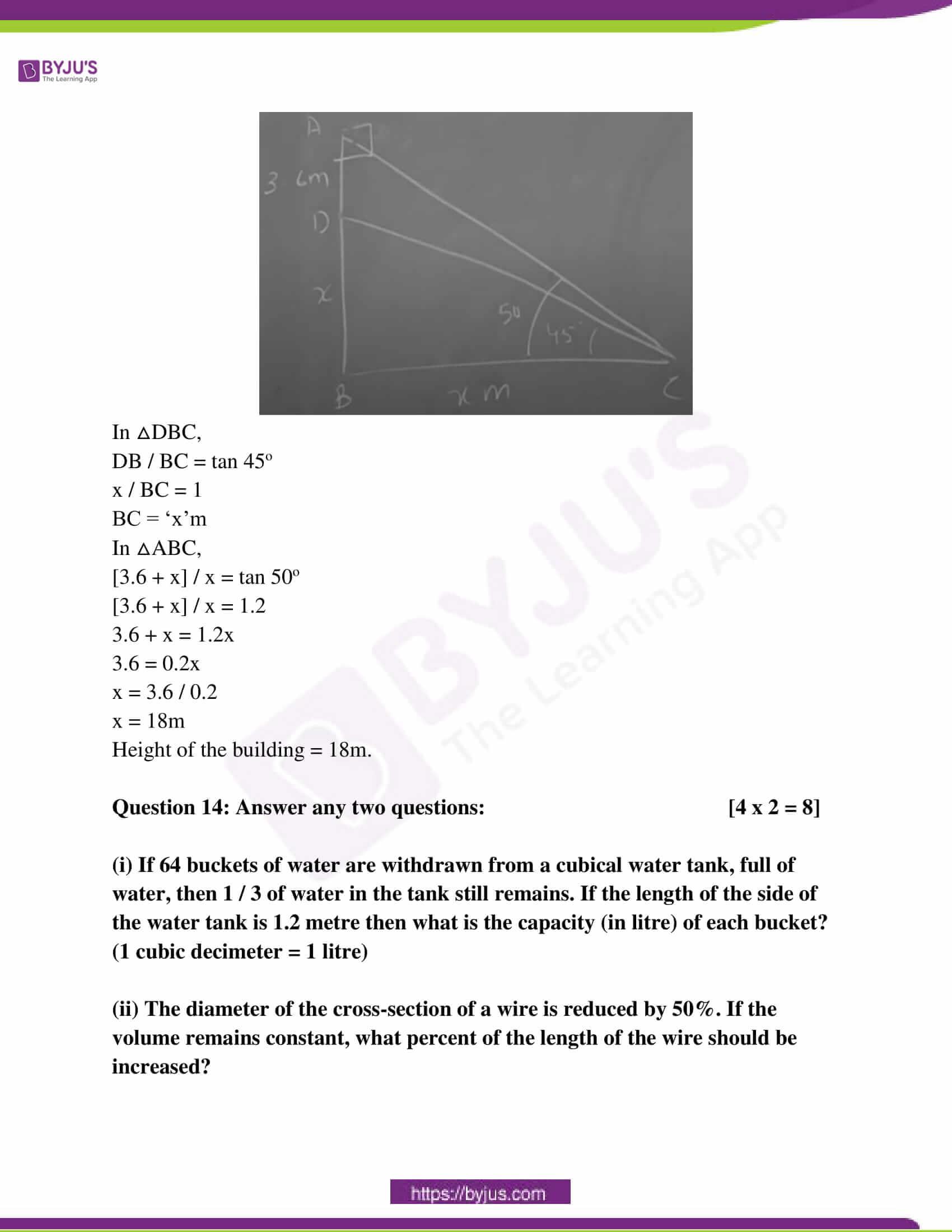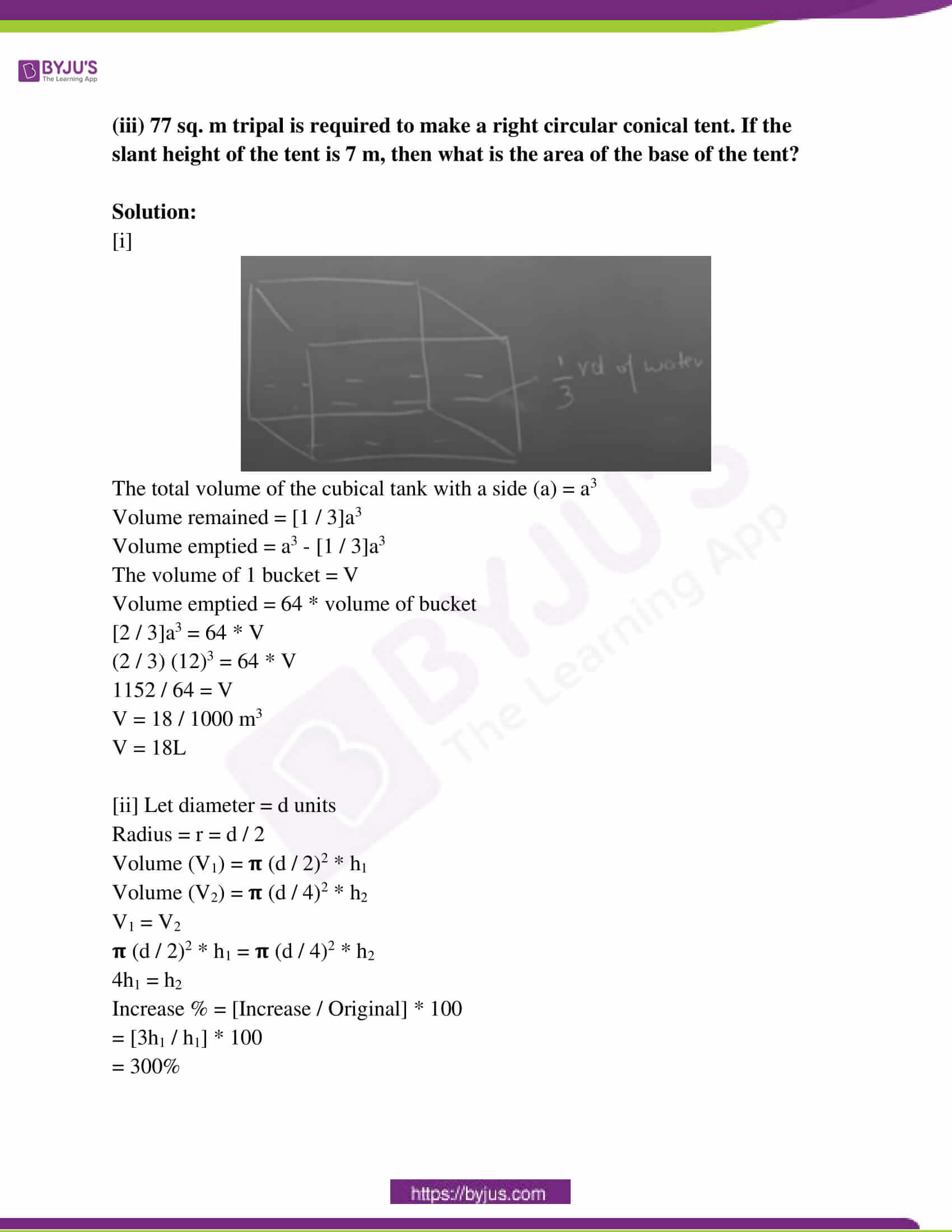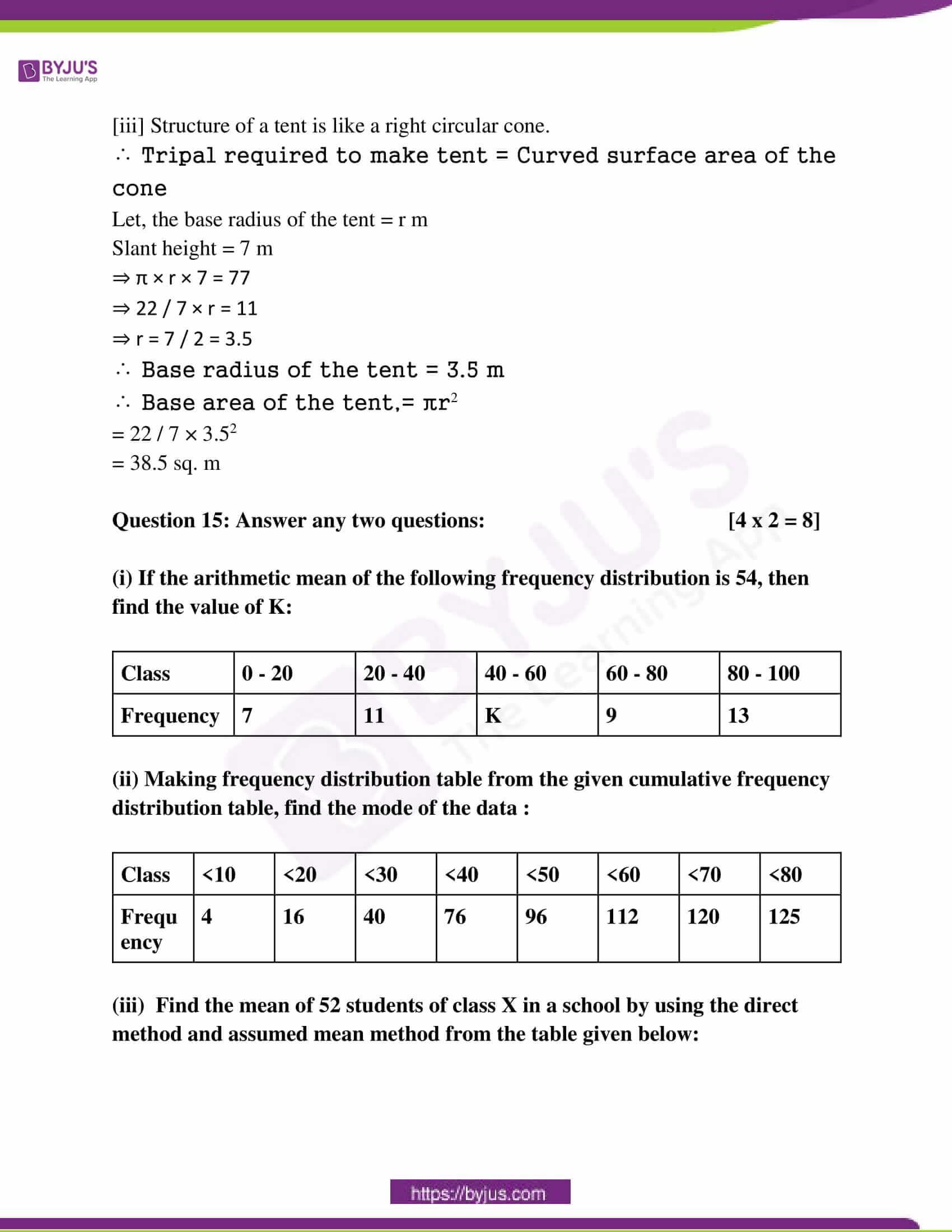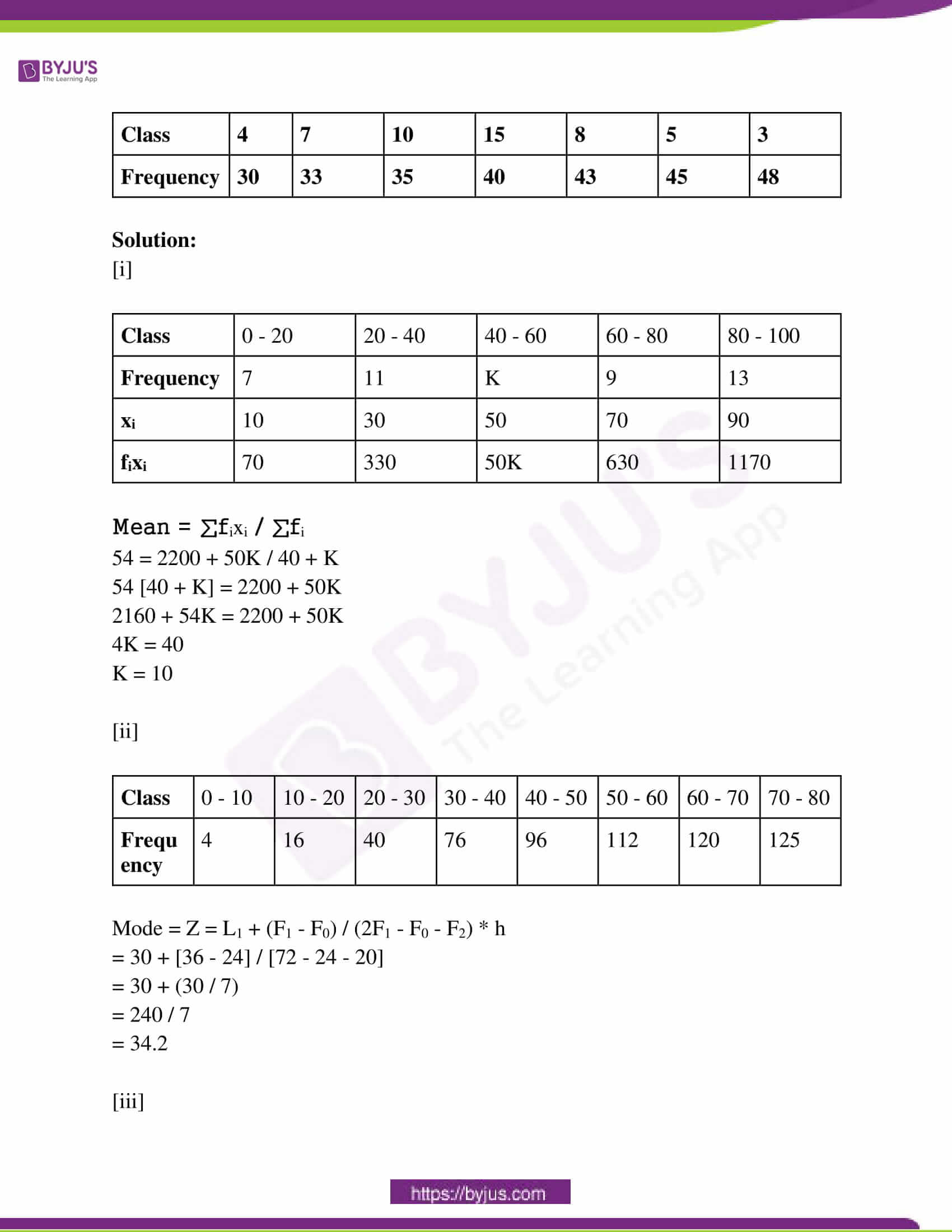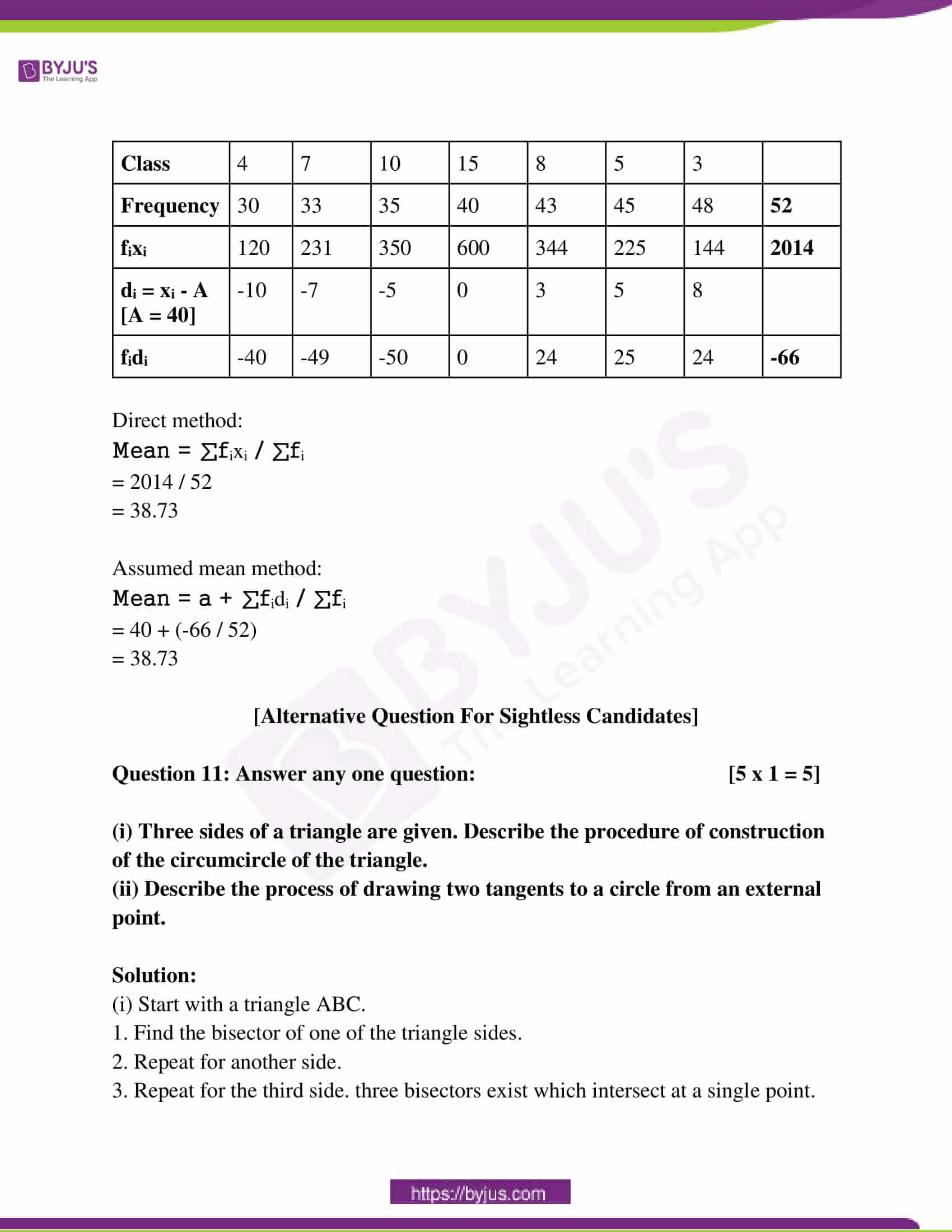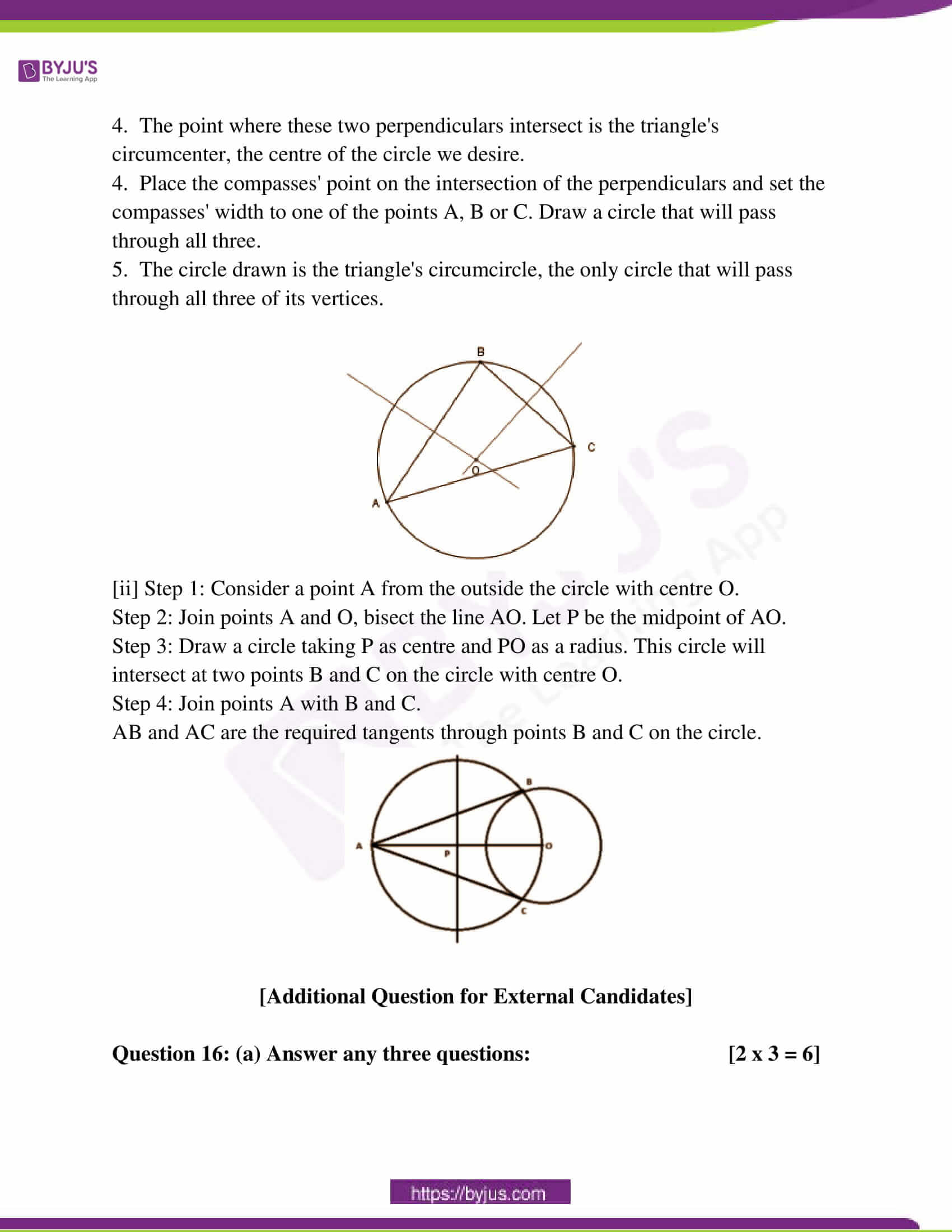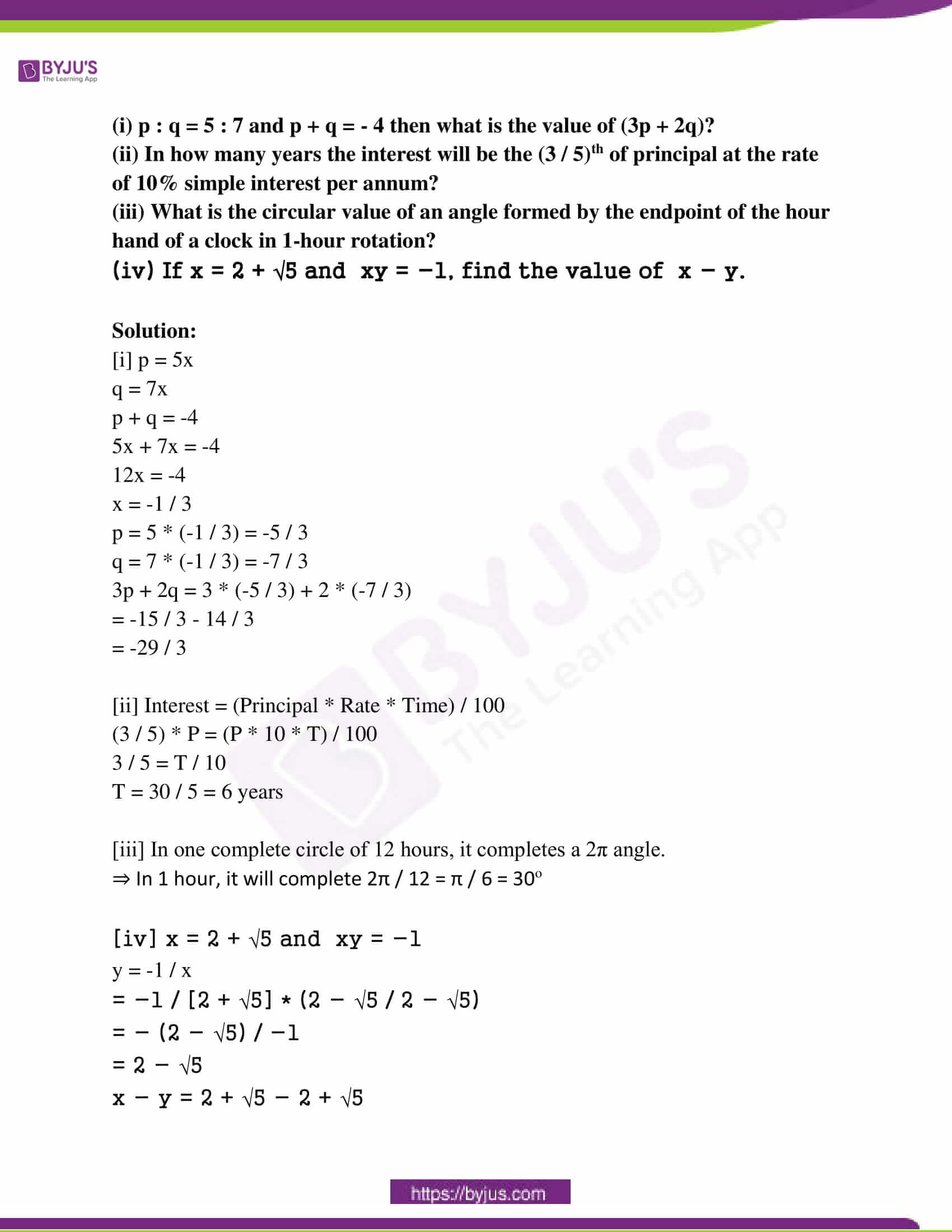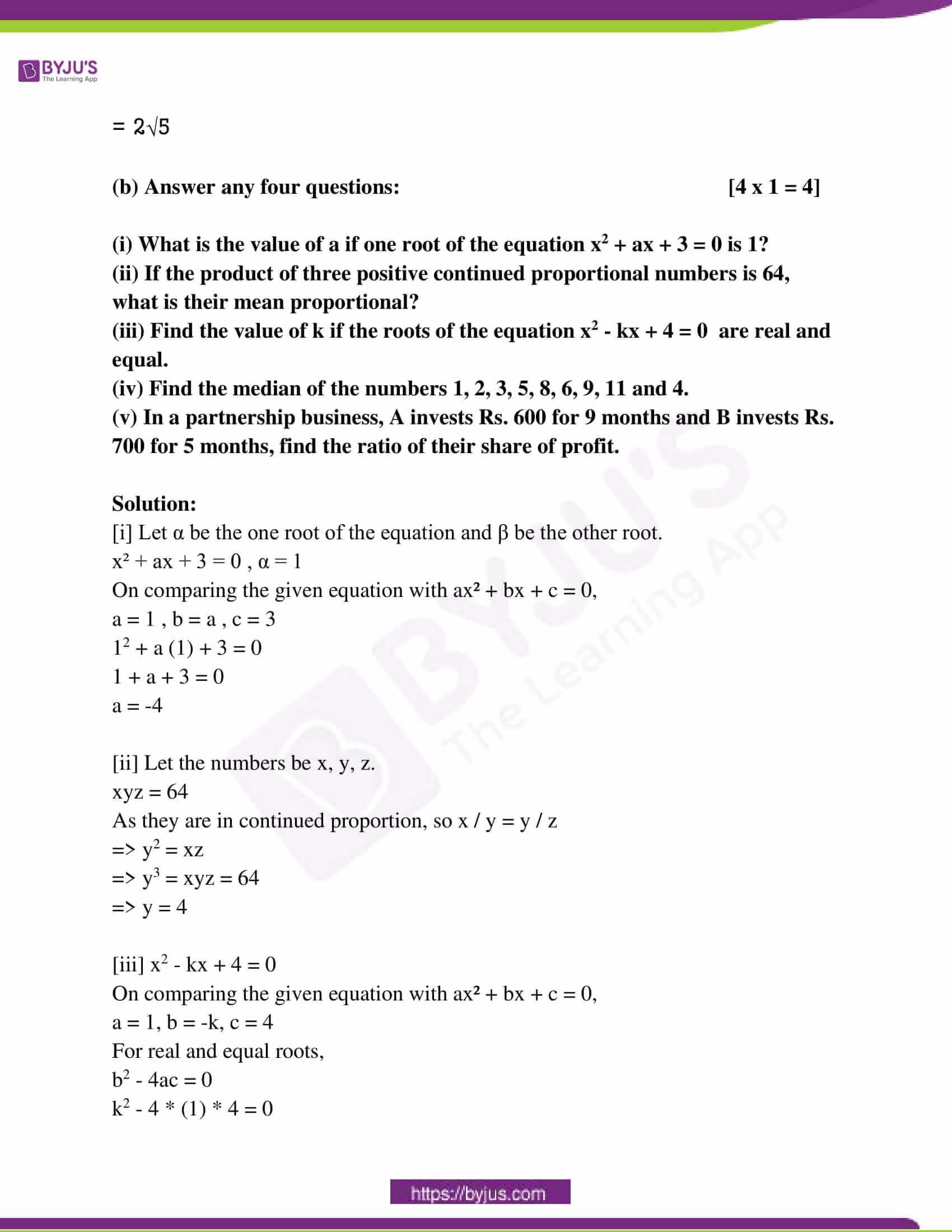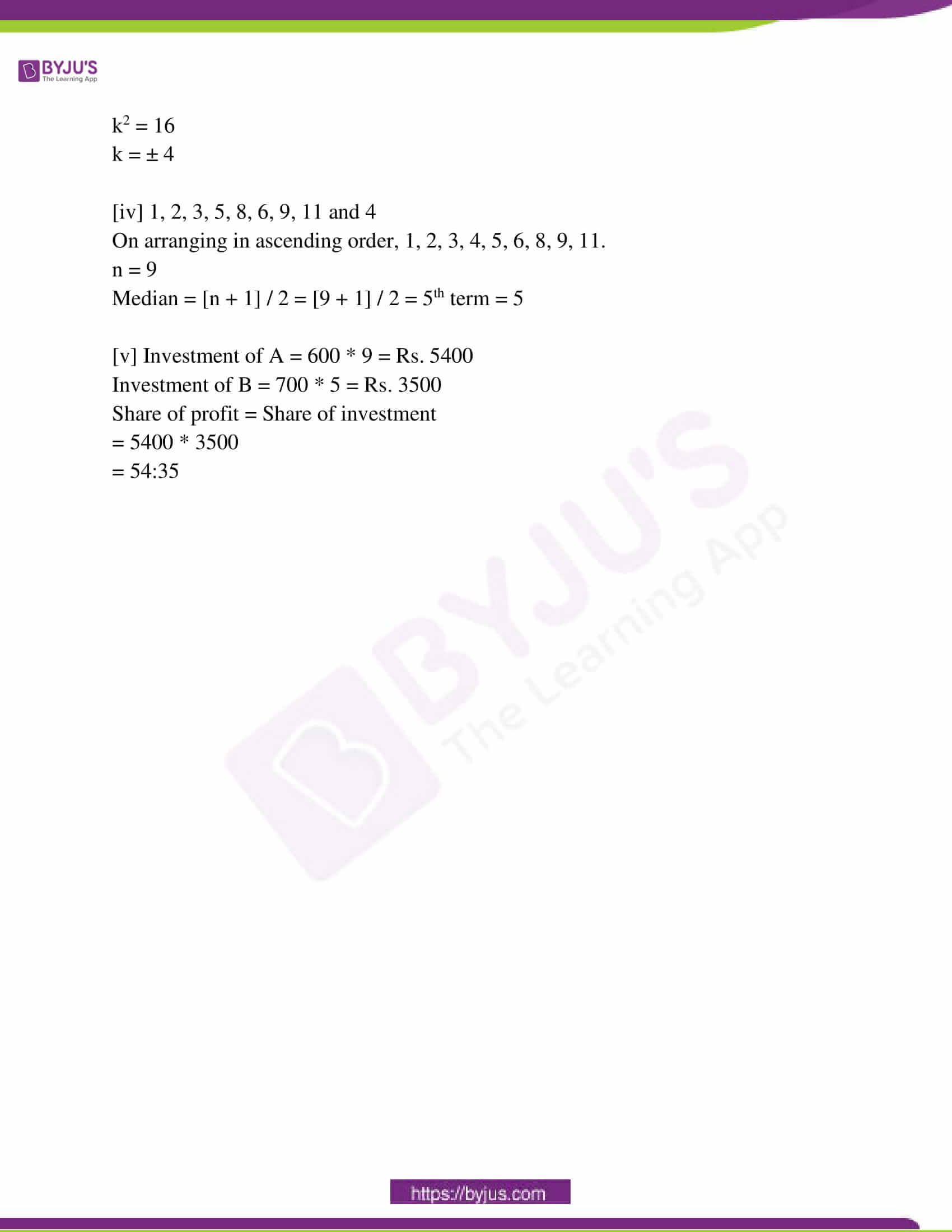Question 1: Choose the correct option in each case from the following questions : [1 x 6 = 6]

[i] In a partnership business, the ratio of the share of profit of two friends is 1 / 2:1 / 3, then the ratio of their principal is –

(a) 2 : 3 (b) 3 : 2 (c) 1 : 1 (d) 5 : 3

1 / 2:1 / 3

3 / 6:2 / 6

3:2

[ii] If p + q = √13 and p – q = √5 then the value of pq is –

(a) 2 (b) 18 (c) 9 (d) 8

p2 + q2 + 2pq = 13

p2 + q2 – 2pq = 5

4pq = 8

pq = 2

[iii] O is the centre of a circle and AB a diameter. ABCD is a cyclic quadrilateral. ∠ABC = 65o, ∠DAC = 40o, then the measure of ∠BCD is –

(a) 75° (b) 105° (c) 115° (d) 80°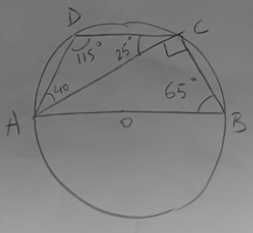∠BCD = 25 + 90 = 115o

[iv] If tan α + cotα = 2, then the value of tan 13 α + cot 13 α is –

(a) 13 (b) 2 (c) 1 (d) 0

tan α + 1 / tan α = 2

tan2 α + 1 – 2 tan α = 0

(tan α – 1)2 = 0

tan α = 1

tan 13 α + cot 13 α

= 1 + 1

= 2

[v] If two cubes of the length of each side 2√6 cm are placed side by side, then the length of the diagonal of the cuboid so produced is –

(a) 10cm (b) 6cm (c) 2cm (d) 12cm

Diagonal = √l2 + b2 + h2

= √(4√6)2 + (2√6)2 + (2√6)2

= √144

= 12

[vi] The mean of the data x1, x2, x3 …… x10 is 20 then the mean of x1 + 4, x2 + 4, x3 + 4, ………. x10 + 4 will be －

(a) 20 (b) 24 (c) 40 (d) 10

Question 2: Fill up the blanks (any five) : [1 x 5 = 5]

(i) A person deposited Rs. 100 in a bank and gets the amount Rs. 121 after two years. The rate of compound interest is _______ %. [Answer: 10%]

P = 100, A = 121, T = 2 years, R = 9

A = P [1 + R / 100]n

121 = 100 [1 + R / 100]2

[11 / 10]2 = [1 + R / 100]2

R = 10%

(ii) If the product and sum of two quadratic surds is a rational number, then surds are _______ surd. [Answer: Conjugate]

(iii) If the bases of two triangles are situated on the same line and the other vertex of the two triangles are common, then the ratio of the areas of two triangles are _______ to the ratio of their bases. [Answer: equal]

(iv) The number of surfaces of a solid right circular cylinder is _______. [Answer: 3]

(v) The simplest value of cos 53o / sin 37o is _______. [Answer: 1]

cos 53o / sin 37o

= sin [90 – 53] / sin 37

= sin 37 / sin 37

= 1

(vi) The variables x1, x2, ….. x100 are in ascending order of their magnitude,

then the median of the variables is _______. [Answer: (x50 + x51) / 2]

Question 3: Write True or False (any five): [1 x 5 = 5]

(i) The difference between the simple interest and the compound interest of Rs. 100 in 1 year at the rate of 10% p.a. is Re. 1. [Answer: False]

(ii) The compound ratio of ab:c2, bc:a and ca:b2 is 1:1. [Answer: True]

(iii) Only one circle can be drawn through three noncollinear points. [Answer: True]

(iv) sin 30o + sin 60o > sin 90o. [Answer: True]

(v) The ratio of the volume of a right circular cone and a right circular cylinder with the same base and height is 1:3. [Answer: True]

(vi) Value of the median of data 2, 3, 9, 10, 9, 3, 9 is 10. [Answer: False]

Question 4: Answer the following questions (any ten) : [2 x 10 = 20]

(i) Find the capital which gives Re. 1 as interest per month at 5% rate of

interest per annum.

Solution:

SI = Re.1

R = 5%

T = 1 month = 1 / 12 years

P = ?

SI = PTR / 100

1 = P * (1 / 12) * 5 / 100

100 = P (5 / 12)

P = Rs.240

(ii) In a partnership business, the ratio of capitals of three men is 3:5:8. The share of profit of the first member is Rs. 60 less than that of the third member, then what is the total profit in the business?

Solution:

The profit will be shared among the partners in their capital ratio.

Let the total profit be X.

1st person profit will be 3X.

2nd person profit will be 5X.

3rd person profit will be 8X.

3X = 8X – 60

60 = 5X

X = 60 / 5

X = 12

Total profit = 3X + 5X + 8X = 16X

Rs. 16 * 12 = Rs. 192 is the total profit.

(iii) If a / 2 = b / 3 = c / 4 = [2a – 3b + 4c] / p, then find p.

Solution:

Let a / 2 = b / 3 = c / 4 = 2a – 3b + 4c / k = m

So a = 2k

b = 3k

c = 4k

2a – 3b + 4c = pk

2 * 2k – 3 * 3k + 4 * 4k = pk

11k = pk

p = 11

(iv) x α y2 and y = 2a when x = a, then show that y2 = 4ax.

Solution:

x α y2

x = ky2 —- (1)

a = k * (2a)2

a = 4a2k

k = 1 / 4a

Put the value of k in (1)

x = (1 / 4a) * y2

4ax = y2

(v) In a trapezium ABCD, BC || AD and AD = 4 cm. The two diagonals AC and BD intersect at the point O in such a way that AO / OC = DO / OB = 1 / 2. Calculate the length of BC.

Solution: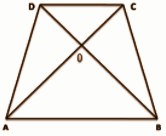ABCD is a trapezium such that BC || AD and AD = 4 cm.

If the diagonals AC and BD intersect at Ο such that AΟ / ΟC = DΟ / ΟB = 1 / 2

Now from the triangle ΔAOB and ΔCOD,

∠AOB = COD (vertically opposite angle)

AO / OC = DO / OB (Given)

From SAS congruence criterion,

ΔAOB ≅ ΔCOD

AO / OC = BO / OD = AB / DC (since corresponding sides of similar triangles are proportional)

=> 1 / 2 = 4 / DC

=> DC = 4 * 2

=> DC = 8 cm

(vi) Two chords AB and AC of a circle are mutually perpendicular to each other. If AB = 4 cm and AC = 3 cm, find the length of the radius of the circle.

Solution: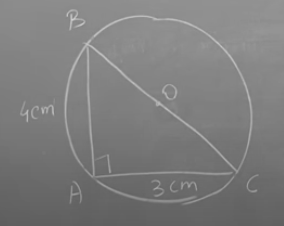The angle of a semicircle is 90o.

BAC is the semi-circle in the figure.

BC is the diameter.

Triangle ABC is a right-angled triangle.

By applying the Pythagoras theorem,

AB2 + AC2 = BC2

42 + 32 = BC2

BC = √16 + 9 = √25

BC = 5cm

= 5 / 2

= 2.5cm

(vii) In △ABC, ∠ABC = 90o and BD ⟂ AC, if AB = 5cm, BC = 12 cm, then find the length of BD.

Solution: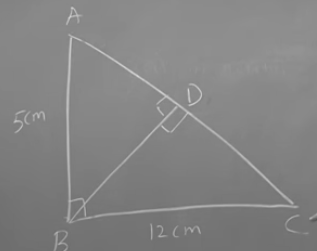In △ABC,

AB2 + BC2 = AC2

52 + 122 = AC2

25 + 144 = AC2

AC = 13cm

Let BD = y, AD = x

CD = 13 – x

x2 + y2 = 52

y2 = 25 – x2 —- (1)

In △BDC,

(13 – x)2 + y2 = 122 —- (2)

(13 – x)2 + (25 – x2) = 144

132 + x2 – 26x + 25 – x2 = 144

169 – 26x + 25 = 144

194 – 144 = 26x

50 / 26 = x

x = 25 / 13

y2 = 25 – (25 / 13)2

= 25 – [625 / 169]

= 3600 / 169

BD = y = 60 / 13 cm

(viii) Find the value(s) of Θ (0o ≤ Θ ≤ 90o) for which 2 sin Θ cos Θ = cos Θ.

Solution:

2 sin Θ cos Θ = cos Θ

2 sin Θ = 1

sin Θ = 1 / 2

Θ = 30o

(ix) If sin 10Θ = cos 8 Θand 10Θ is a positive acute angle, then find the value of tan 9Θ.

Solution:

sin 10Θ° = cos 8Θ°

sin 10Θ° = sin (90 – 8Θ)°

Comparing both sides:

10Θ = 90 – 8Θ

18Θ = 90

Θ = 90 / 18

Θ = 5°

Therefore,

tan 9Θ° = tan 9 × 5°

= tan 45°

= 1

(x) The length, breadth and height of a cuboidal room be a unit, b unit, and c unit respectively and a + b + c = 25, ab + bc + ca = 240.5 then find the length of the longest rod to be kept inside the room.

Solution:

The diagonal of the cuboidal = √a2 + b2 + c2 units

(a + b + c)² = a² + b² + c² + 2(ab + bc + ca)

⇒ (25)² = (a² + b² + c²) + 2 (240.5) {Since, (a + b + c) = 25 and (ab + bc + ca) = 240.5. given}

⇒ (a² + b² + c²) = 144

⇒ √a2 + b2 + c2

= √144

= 12 units

(xi) The area of the curved surface of a right circular cone is √5 times that of the base of the cone, find the ratio of the height and the radius of the base of the cone.

Solution:

The area of the curved surface of a right circular cone is √5 times its area of the base.

Area of the curved surface of a right circular cone = πRL

L = Slant Height

Area of Base = πR²

Area of the curved surface of a right circular cone = √5 * Area of Base

=> πRL = √5 πR²

=> L = √5 R

L² = H² + R² ( H = Height of cone)

=> (√5 R)² = H² + R²

=> 5R² = H² + R²

=> H² = 4R²

=> H = 2R

The height of the cone is 2 times its radius.

(xii) The mid-value of the first (2n + 1) consecutive natural number is [n + 103] / 3. Find n.

Solution:

The first (2n + 1) consecutive natural numbers form an arithmetic progression.

1, 2, 3, 4……………….. (2n + 1)

As (2n + 1) is odd number, the middle term will be (2n + 2) / 2 = (n + 1)th term

Now, value of Tn+1 term is given by

= 1 + (n + 1 – 1)*1

= n + 1

n + 1 = (n + 103) / 3

3n + 3 = n + 103

3n – n = 103 – 3

2n = 100

n = 100 / 2 = 50

Therefore the value of n = 50.

Question 5: Answer any one question : [5 x 1 = 5]

(i) If interest is compounded half-yearly what will be the compound interest and amount on Rs. 8,000 at the rate of 10% compound interest per annum for 1 (1 / 2) years?

(ii) Two friends start a partnership business investing Rs. 40,000 and Rs. 50,000 respectively. There is an agreement between them that 50% of the profit will be divided equally and the rest amount of profit will be distributed between them in the ratio of their principal. If the share of profit of 1st friend is Rs. 800 less than that of the 2nd friend, find the share of profit of the lst friend.

Solution:

[i] P = Rs. 8000

R = 10%

T = 1 (1 / 2) years = 3 / 2 years

Compounded half-yearly, R = 10 / 2 = 5%

T = 2 * (3 / 2) = 3 years

A = P {1 + (R / 100)}n

A = 8000 {1 + (5 / 100)}3

= 8000 * (1.05)3

= Rs. 9261

CI = A – P

= 9261 – 8000

= Rs. 1261

[ii] Let say Total Profit Share = P

Profit Share of 1st friend = (50 / 100)(P/2) + (40000 / 90000)(P / 2)

= P / 4 + 4P / 18

Profit Share of 2nd friend = (50 / 100)(P / 2) + (50000 / 90000)(P / 2)

= P / 4 + 5P / 18

P / 4 + 5P / 18 – (P / 4 + 4P / 18) = P / 18

P / 18 = 800

=> P = 14400 Rs

First friend share = 14400 / 4 + 4 * 14400 / 18

= 3600 + 3200

= 6800 Rs

2nd friend share = 14400 / 4 + 5 * 14400 / 18

= 3600 + 4000

= 7600 Rs

Question 6: Solve any one question: [3 x 1 = 3]

(i) Determine the equation whose roots are the square of the roots of the equation x2 + x + 1 = 0.

(ii) If the price of 1 dozen pens is reduced by Rs 6, then 3 more pens will be bought at Rs 30. calculate the price of one dozen pens before the reduction of price.

Solution:

[i] x2 + x + 1 = 0

ax2 + bx + c = 0

a = 1, b = 1, c = 1

ɑ + β = -b / a = -1 / 1 = 1

ɑ β = c / a = 1 / 1 = 1

(ɑ + β)2 = ɑ2 + β2 + 2 ɑ β

(-1)2 = ɑ2 + β2 + 2 * 1

-1 = ɑ2 + β2 — (1)

ɑ2 β2 = 1 —- (2)

x2 – (ɑ2 + β2) x + ɑ2 β2

x2 + x + 1 = 0

[ii] Let the price of 1 dozen pens before reduction be x.

After the reduction, the price of 12 pens is x – 6.

Price of 15 pens = ([x – 6] / 12) * 15 —– (1)

Price of 15 pens = Rs. 30 —- (2)

([x – 6] / 12) * 15 = 30

[x – 6] / 12 = 2

x – 6 = 24

x = Rs. 30

Question 7: Answer any one question: [3 x 1 = 3]

(i) Simplify: [4√3 / 2 – √2] – [30 / 4√3 – √18] – [√18 / 3 – √12].

(ii) If [(1 / x) – (1 / y)] α [1 / x – y], then show that x2 + y2 α xy.

Solution:

[i]  = (4√3) / (2 – √2) = (4√3) (2 + √2) / (2 – √2) (2 + √2)

= (8√3 + 4√6) / 2

= 4√3 + 2√6 …………………(1)

 = 30 / (4√3 – √18)

= 30 * (4√3 + √18) / (4√3 – √18) (4 √3 + √18)

= (120√3 + 30 √18) / (48 – 18)

= 30 (4√3 + √18) / 30

= 4√3 + 3√2 ………………(2)

 = √18 / (3 – 2√3)

= √18 (3 + 2√3) / (3 – 2√3) (3 + 2√3)

= (3√18 + 2√54) / 9 – 12

= (9√2 + 6√6) / -3

= -3√2 -2√6 ………………(3)

Now by combining all 3 terms:

(4√3 + 2√6) — (4√3 + 3√2) — (-3√2 – 2√6)

= 4√3 + 2√6 – 4√3 – 3√2 + 3√2 + 2√6

= 2√6 + 2√6

= 4√6

[ii] [y – x] / xy = k * [1 / [x – y]]

(y – x) (x – y) = k* xy

xy – x2 – y2 – xy = k * xy

x2 + y2 = 2xy – kxy

x2 + y2 = (2 – k) xy

x2 + y2 α xy

Question 8: Answer any one question: [3 x 1 = 3]

(i) If (3x – 2y) : (x + 3y) = 5 : 6, then find the value of (2x + 5y) : (3x + 4y).

(ii) If [b + c – a] / [y + z – x] = [c + a – b] / [z + x – y] = [a + b – c] / [x + y – z], then prove that [a / x] = [b / y] = [c / z].

Solution:

[i] (3x – 2y) : (x + 3y) = 5 : 6

6 * (3x – 2y) = 5 * (x + 3y)

18x – 12y = 5x + 15y

18x – 5x = 15y + 12y

13x = 27y

x = [27 / 13] * y

(2x + 5y) / (3x + 4y)

= (2 * [27y / 13] + 5y) / (3 * [27y / 13] + 4y)

= ([54y / 13] + 5y) / [(81y / 13) + 4y]

= 119y / 133y

= 119 / 133

= 17 / 19

= 17:19

[ii] [b + c – a] = k [y + z – x] —- (1)

[c + a – b] = k [z + x – y] —- (2)

[a + b – c] = k [x + y – z] —- (3)

a + b + c = k (x + y + z) —- (4)

Subtract (1) from (4),

2a = k (2x)

a / x = k

Similarly,

b / y = k

c / z = k

From the above equations,

[a / x] = [b / y] = [c / z]

Question 9: Answer any one question: [5 x 1 = 5]

(i) Prove that the semicircular angle is a right angle.

(ii) If two circles touch each other externally then the point of contact will lie on the line segment joining the two centres – Prove it.

Solution:

[i] Now POQ is a straight line passing through centre O.

∴ Angle subtended by arc PQ at O is

∠POQ = 180°

The angle subtended by an arc at the centre is double the angle subtended by any point on the remaining part of the circle.

∴∠POQ = 2 ∠PAQ

∠POQ / 2 = ∠PAQ

180 / 2 = ∠PAQ

∠PAQ = 90o

[ii] Two circles are touching each other externally in the figure on the left side whereas, on the right side, two circles are touching each other internally. At the point of contact, a common tangent L can be drawn to both the circles: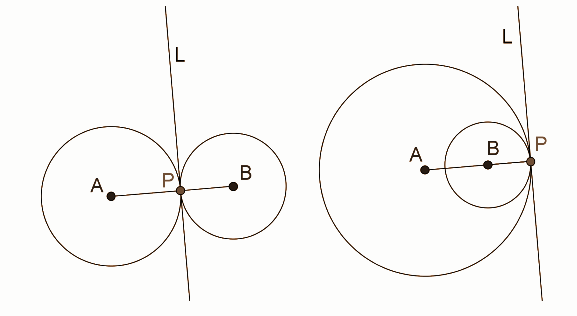In both the cases above, AP must be perpendicular to L, and so must BP. This is because L is the tangent to both the circles at P. Thus, both the perpendiculars AP and BP pass through the same point P, which necessarily implies that A, P and B are collinear.

Question 10: Answer any one question: [3 x 1 = 3]

(i) If a quadrilateral ABCD is circumscribed about a circle with centre O, prove that AB + CD = BC + DA.

(ii) If in ΔABC, ∠A is the right angle and BP and CQ are two medians, then prove that, 5BC2 = 4(BP2 + CQ2).

Solution:

[i]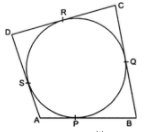Since the tangents drawn from an exterior point to a circle are equal in length.

∴ AP = AS …(i)

BP = BQ …(ii)

CR = CQ …(iii) and

DR = DS …(iv)

Adding (i), (ii), (iii) and (iv),

AP + BP + CR + DR = AS + BQ + CQ + DS

⇒ (AP + BP) + (CR + DR)

= (AS + DS) + (BQ + CQ)

⇒ AB + CD = AD + BC

Hence, AB + CD = BC + DA.

[ii]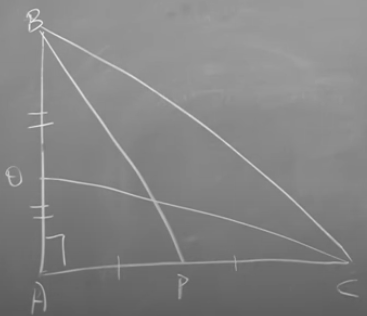In △ABC,

AB2 + AC2 = BC2 —- (1)

In △BAP,

AB2 + AP2 = BP2 —- (2)

In △QAC,

QA2 + AC2 = QC2 —- (3)

LHS = 5BC2 = 5 (AB2 + AC2)

RHS = 4 [AB2 + (AC / 2)2 + AC2 (AB / 2)2]

= 4 [5 (AB2 + AC2) / 4]

= 5 [AB2 + AC2]

Question 11: Answer any one question: [5 x 1 = 5]

(i) Draw a triangle ABC of which BC = 7 cm, AB = 5 cm and AC = 6 cm. Then draw the circumcircle of ΔABC. (Only traces of construction are required)

(ii) Construct a circle of radius 4 cm and draw two tangents to the circle from an external point at a distance of 6.5 cm from the centre of the circle.

Solution:

[i]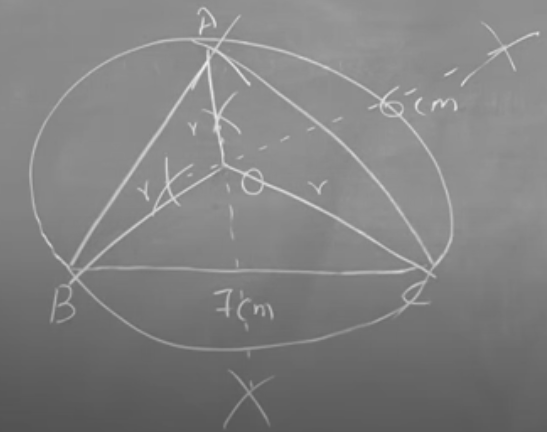[ii]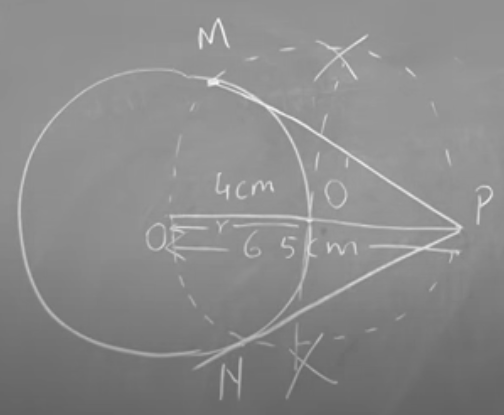Question 12: Answer any two questions : [3 x 2 = 6]

[i] In △ABC, ∠C = 90o, if BC = m and AC = n then prove that, m sin A + n sin B = √m2 + n2.

[ii] Find the value of (4 / 3) cot2 30o + 3 sin2 60o – 2 cosec2 60o – (3 / 4) tan2 30o.

[iii] If ∠P + ∠Q = 90° then show that √sin P / cos Q – (sin P cos Q) = cos2 P.

Solution:

[i]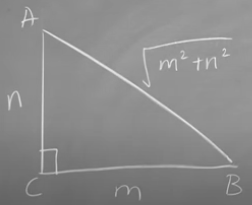In △ACB,

AC2 + BC2 = AB2

n2 + m2 = AB2

AB = √m2 + n2

LHS = m sin A + n sin B

= m * (m / √m2 + n2) + n * (n / √m2 + n2)

= m2 / √m2 + n2 + n2 / √m2 + n2

= m2 + n2 / √m2 + n2

= √m2 + n2 * √m2 + n2 / √m2 + n2

= √m2 + n2

= RHS

[ii] (4 / 3) cot2 30o + 3 sin2 60o – 2 cosec2 60o – (3 / 4) tan2 30o

= (4 / 3) * (√3)2 + 3 * (√3 / 2)2 – 2 * (2 / √3)2 – (3 / 4) * (1 / √3)2

= (4 / 3) * (3) + 3 * (3 / 4) – 2 * (4 / 3) – (3 / 4) * (1 / 3)

= 4 + 9 / 4 – 8 / 3 – 3 / 12

= 10 / 3

[iii] ∠P + ∠Q = 90°

90 – Q = P

LHS = √sin P / cos Q – (sin P cos Q)

= √sin P / sin (90 – Q) – sin P (sin [90 – Q])

= √sin P / sin P – sin P sin P

= 1 – sin2 P

= cos2 P

Question 13: Answer any one question: [5 x 1 = 5]

(i) From a quay of a river, 600 metres wide, two boats start in two different directions to reach the opposite side of the river. The first boat moves to make an angle of 30° with this bank and the second boat moves to make an angle 90° with the direction of the first boat. What will be the distance between the two boats when both of them reach the other side?

(ii) The length of the flag post at the roof of a three-storied building is 3.6 metre. The angles of elevation of the top and foot of the post are 50° and 45° respectively from a point on the road. Find the height of the building. [Take tan 50° = 1.2]

Solution:

[i]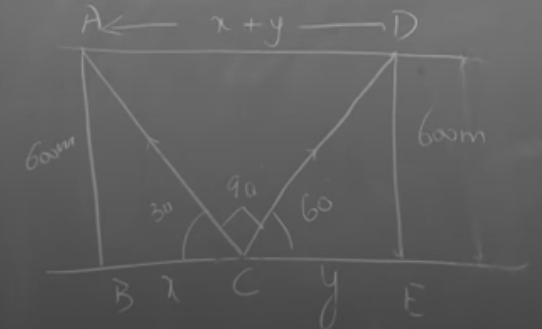In △ABC,

tan 30o = 600 / x

1 / √3 = 600 / x

x = 600√3

In △DEC,

tan 60o = 600 / y

√3 = 600 / y

y = 600 / √3

x + y = 600√3 + 600 / √3

= [1800 + 600] / √3

= 2400 / √3

= 800√3m

[ii]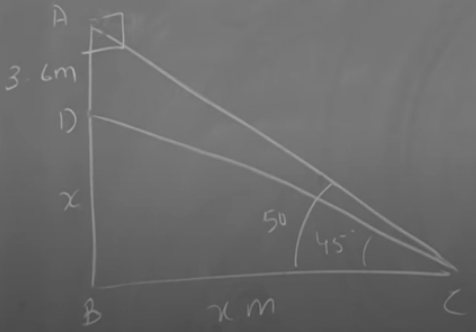In △DBC,

DB / BC = tan 45o

x / BC = 1

BC = ‘x’m

In △ABC,

[3.6 + x] / x = tan 50o

[3.6 + x] / x = 1.2

3.6 + x = 1.2x

3.6 = 0.2x

x = 3.6 / 0.2

x = 18m

Height of the building = 18m.

Question 14: Answer any two questions: [4 x 2 = 8]

(i) If 64 buckets of water are withdrawn from a cubical water tank, full of water, then 1 / 3 of water in the tank still remains. If the length of the side of the water tank is 1.2 metre then what is the capacity (in litre) of each bucket? (1 cubic decimeter = 1 litre)

(ii) The diameter of the cross-section of a wire is reduced by 50%. If the volume remains constant, what percent of the length of the wire should be increased?

(iii) 77 sq. m tripal is required to make a right circular conical tent. If the slant height of the tent is 7 m, then what is the area of the base of the tent?

Solution:

[i]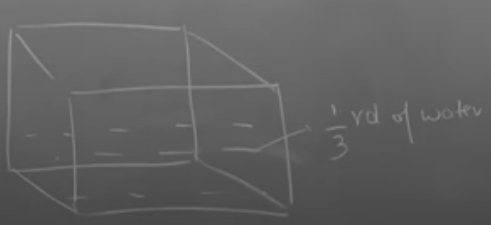The total volume of the cubical tank with a side (a) = a3

Volume remained = [1 / 3]a3

Volume emptied = a3 – [1 / 3]a3

The volume of 1 bucket = V

Volume emptied = 64 * volume of bucket

[2 / 3]a3 = 64 * V

(2 / 3) (12)3 = 64 * V

1152 / 64 = V

V = 18 / 1000 m3

V = 18L

[ii] Let diameter = d units

Radius = r = d / 2

Volume (V1) = 𝛑 (d / 2)2 * h1

Volume (V2) = 𝛑 (d / 4)2 * h2

V1 = V2

𝛑 (d / 2)2 * h1 = 𝛑 (d / 4)2 * h2

4h1 = h2

Increase % = [Increase / Original] * 100

= [3h1 / h1] * 100

= 300%

[iii] Structure of a tent is like a right circular cone.

∴ Tripal required to make tent = Curved surface area of the cone

Let, the base radius of the tent = r m

Slant height = 7 m

⇒ π × r × 7 = 77

⇒ 22 / 7 × r = 11

⇒ r = 7 / 2 = 3.5

∴ Base radius of the tent = 3.5 m

∴ Base area of the tent,= πr2

= 22 / 7 × 3.52

= 38.5 sq. m

Question 15: Answer any two questions: [4 x 2 = 8]

(i) If the arithmetic mean of the following frequency distribution is 54, then find the value of K:

 Class 0 – 20 20 – 40 40 – 60 60 – 80 80 – 100 Frequency 7 11 K 9 13

(ii) Making frequency distribution table from the given cumulative frequency distribution table, find the mode of the data :

 Class <10 <20 <30 <40 <50 <60 <70 <80 Frequency 4 16 40 76 96 112 120 125

(iii) Find the mean of 52 students of class X in a school by using the direct method and assumed mean method from the table given below:

 Class 4 7 10 15 8 5 3 Frequency 30 33 35 40 43 45 48

Solution:

[i]
 Class 0 – 20 20 – 40 40 – 60 60 – 80 80 – 100 Frequency 7 11 K 9 13 xi 10 30 50 70 90 fixi 70 330 50K 630 1170

Mean = ∑fixi / ∑fi

54 = 2200 + 50K / 40 + K

54 [40 + K] = 2200 + 50K

2160 + 54K = 2200 + 50K

4K = 40

K = 10

[ii]
 Class 0 – 10 10 – 20 20 – 30 30 – 40 40 – 50 50 – 60 60 – 70 70 – 80 Frequency 4 16 40 76 96 112 120 125

Mode = Z = L1 + (F1 – F0) / (2F1 – F0 – F2) * h

= 30 + [36 – 24] / [72 – 24 – 20]

= 30 + (30 / 7)

= 240 / 7

= 34.2

[iii]
 Class 4 7 10 15 8 5 3 Frequency 30 33 35 40 43 45 48 52 fixi 120 231 350 600 344 225 144 2014 di = xi – A [A = 40] -10 -7 -5 0 3 5 8 fidi -40 -49 -50 0 24 25 24 -66

Direct method:

Mean = ∑fixi / ∑fi

= 2014 / 52

= 38.73

Assumed mean method:

Mean = a + ∑fidi / ∑fi

= 40 + (-66 / 52)

= 38.73

[Alternative Question For Sightless Candidates]

Question 11: Answer any one question: [5 x 1 = 5]

(i) Three sides of a triangle are given. Describe the procedure of construction of the circumcircle of the triangle.

(ii) Describe the process of drawing two tangents to a circle from an external point.

Solution:

1. Find the bisector of one of the triangle sides.

2. Repeat for another side.

3. Repeat for the third side. three bisectors exist which intersect at a single point.

4. The point where these two perpendiculars intersect is the triangle’s circumcenter, the centre of the circle we desire.

4. Place the compasses’ point on the intersection of the perpendiculars and set the compasses’ width to one of the points A, B or C. Draw a circle that will pass through all three.

5. The circle drawn is the triangle’s circumcircle, the only circle that will pass through all three of its vertices.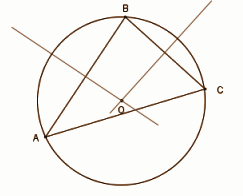[ii] Step 1: Consider a point A from the outside the circle with centre O.

Step 2: Join points A and O, bisect the line AO. Let P be the midpoint of AO.

Step 3: Draw a circle taking P as centre and PO as a radius. This circle will intersect at two points B and C on the circle with centre O.

Step 4: Join points A with B and C.

AB and AC are the required tangents through points B and C on the circle.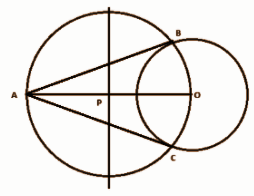Question 16: (a) Answer any three questions: [2 x 3 = 6]

(i) p : q = 5 : 7 and p + q = – 4 then what is the value of (3p + 2q)?

(ii) In how many years the interest will be the (3 / 5)th of principal at the rate of 10% simple interest per annum?

(iii) What is the circular value of an angle formed by the endpoint of the hour hand of a clock in 1-hour rotation?

(iv) If x = 2 + √5 and xy = -1, find the value of x – y.

Solution:

[i] p = 5x

q = 7x

p + q = -4

5x + 7x = -4

12x = -4

x = -1 / 3

p = 5 * (-1 / 3) = -5 / 3

q = 7 * (-1 / 3) = -7 / 3

3p + 2q = 3 * (-5 / 3) + 2 * (-7 / 3)

= -15 / 3 – 14 / 3

= -29 / 3

[ii] Interest = (Principal * Rate * Time) / 100

(3 / 5) * P = (P * 10 * T) / 100

3 / 5 = T / 10

T = 30 / 5 = 6 years

[iii] In one complete circle of 12 hours, it completes a 2π angle.

⇒ In 1 hour, it will complete 2π / 12 = π / 6 = 30o

[iv] x = 2 + √5 and xy = -1

y = -1 / x

= -1 / [2 + √5] * (2 – √5 / 2 – √5)

= – (2 – √5) / -1

= 2 – √5

x – y = 2 + √5 – 2 + √5

= 2√5

(b) Answer any four questions: [4 x 1 = 4]

(i) What is the value of a if one root of the equation x2 + ax + 3 = 0 is 1?

(ii) If the product of three positive continued proportional numbers is 64, what is their mean proportional?

(iii) Find the value of k if the roots of the equation x2 – kx + 4 = 0 are real and equal.

(iv) Find the median of the numbers 1, 2, 3, 5, 8, 6, 9, 11 and 4.

(v) In a partnership business, A invests Rs. 600 for 9 months and B invests Rs. 700 for 5 months, find the ratio of their share of profit.

Solution:

[i] Let α be the one root of the equation and β be the other root.

x² + ax + 3 = 0 , α = 1

On comparing the given equation with ax² + bx + c = 0,

a = 1 , b = a , c = 3

12 + a (1) + 3 = 0

1 + a + 3 = 0

a = -4

[ii] Let the numbers be x, y, z.

xyz = 64

As they are in continued proportion, so x / y = y / z

=> y2 = xz

=> y3 = xyz = 64

=> y = 4

[iii] x2 – kx + 4 = 0

On comparing the given equation with ax² + bx + c = 0,

a = 1, b = -k, c = 4

For real and equal roots,

b2 – 4ac = 0

k2 – 4 * (1) * 4 = 0

k2 = 16

k = ± 4

[iv] 1, 2, 3, 5, 8, 6, 9, 11 and 4

On arranging in ascending order, 1, 2, 3, 4, 5, 6, 8, 9, 11.

n = 9

Median = [n + 1] / 2 = [9 + 1] / 2 = 5th term = 5

[v] Investment of A = 600 * 9 = Rs. 5400

Investment of B = 700 * 5 = Rs. 3500

Share of profit = Share of investment

= 5400 * 3500

= 54:35